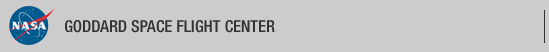+ Visit NASA.gov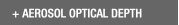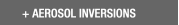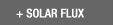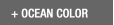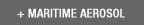Web Site Feature AERONET Data Synergy Tool - Access Earth Science data sets for AERONET sites+Home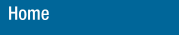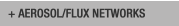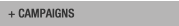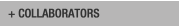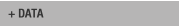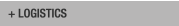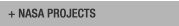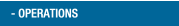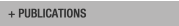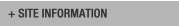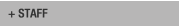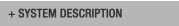AERONET DATA ACCESS

AERONET Site Lists (V3)

+ All Lists# Report of DCP transmissions

 Status Legend

AERONET No 6 [1317E33A] - 25 msg rcvd (12284 b) site : GSFC (Last Tx is before schedule by 0 min 3 sec
25 messages stepped outside their assigned time window)
AERONET No 26 [1316E1C0] - 25 msg rcvd (17344 b) site : GSFC (Last Tx is late by 0 min 1 sec)
AERONET No 28 [13101F58] - 25 msg rcvd (19856 b) site : White_Sands_HELSTF (Last Tx is precisely on time)
AERONET No 59 [131A03AE] - 25 msg rcvd (3749 b) site : Sevilleta (Last Tx is precisely on time)
AERONET No 134  - 24 msg rcvd (25357 b) site : GSFC (Last Tx is before schedule by 0 min 3 sec
24 messages stepped outside their assigned time window , 1b)
AERONET No 163  - 25 msg rcvd (17062 b) site : UCSB (Last Tx is precisely on time)
AERONET No 196 [1319A458] - 25 msg rcvd (19945 b) site : Rio_Branco (Last Tx is precisely on time)
AERONET No 306 [131A40A4] - 25 msg rcvd (9564 b) site : Easton-MDE (Last Tx is precisely on time , 25b)
AERONET No 307 [1318152C] - 25 msg rcvd (6477 b) site : Ames (Last Tx is late by 0 min 1 sec , 4b)
AERONET No 317 [131982B4] - 25 msg rcvd (8433 b) site : Tudor_Hill (Last Tx is precisely on time)
AERONET No 319 [131820B6] - 25 msg rcvd (17413 b) site : Trelew (Last Tx is late by 0 min 1 sec 49s)
AERONET No 330 [131481D2] - 25 msg rcvd (23023 b) site : GSFC (Last Tx is late by 0 min 1 sec)
AERONET No 365 [131A53D2] - 24 msg rcvd (11700 b) site : Ji_Parana_SE (Last Tx is precisely on time , 15b)
AERONET No 374 [131713BE] - 25 msg rcvd (22954 b) site : TABLE_MOUNTAIN_CA (Last Tx is precisely on time)
AERONET No 409 [131AA356] - 25 msg rcvd (15702 b) site : Mingo (Last Tx is precisely on time)
AERONET No 601 [1319E752] - 25 msg rcvd (2800 b) site : CCNY ()
AERONET No 635 [1319D2C8] - 25 msg rcvd (14447 b) site : Alta_Floresta (Last Tx is precisely on time)
AERONET No 813 [1310118A] - 25 msg rcvd (27185 b) site : Yuma (Last Tx is precisely on time)

# Aeronet No 6 [1317E33A] (GSFC)

Last Tx is before schedule by 0 min 3 sec
25 messages stepped outside their assigned time window

12:12:2019,13:41:47 (UTC) 'G' (DCP total: 673) Cimel : 6 time corr. = -29 sec (DCP Volt = 13.00 Lat= 38.993 Long= -76.840(0.0 km from GSFC)) staD nsu staD nsu staD nsu staD nsu staD nsu
12:12:2019,22:41:47 (UTC) 'G' (DCP total: 777) Cimel : 6 time corr. = -29 sec (DCP Volt = 13.00 Lat= 38.993 Long= -76.840(0.1 km from GSFC)) nsu staD nsu staD nsu staD nsu staD nsu staD nsu staD
12:12:2019,23:41:47 (UTC) 'G' (DCP total: 87) Cimel : 6 time corr. = -28 sec (DCP Volt = 13.00 Lat= 38.993 Long= -76.840(0.1 km from GSFC))
13:12:2019,00:41:47 (UTC) 'G' (DCP total: 87) Cimel : 6 time corr. = -28 sec (DCP Volt = 13.00 Lat= 38.993 Long= -76.840(0.0 km from GSFC))
13:12:2019,01:41:47 (UTC) 'G' (DCP total: 87) Cimel : 6 time corr. = -30 sec (DCP Volt = 13.00 Lat= 38.993 Long= -76.840(0.0 km from GSFC))
13:12:2019,02:41:47 (UTC) 'G' (DCP total: 87) Cimel : 6 time corr. = -30 sec (DCP Volt = 13.00 Lat= 38.993 Long= -76.840(0.0 km from GSFC))
13:12:2019,03:41:47 (UTC) 'G' (DCP total: 87) Cimel : 6 time corr. = -29 sec (DCP Volt = 13.00 Lat= 38.992 Long= -76.840(0.0 km from GSFC))
13:12:2019,04:41:47 (UTC) 'G' (DCP total: 87) Cimel : 6 time corr. = -30 sec (DCP Volt = 13.00 Lat= 38.993 Long= -76.840(0.0 km from GSFC))
13:12:2019,05:41:47 (UTC) 'G' (DCP total: 87) Cimel : 6 time corr. = -30 sec (DCP Volt = 13.00 Lat= 38.993 Long= -76.841(0.1 km from GSFC))
13:12:2019,06:41:47 (UTC) 'G' (DCP total: 87) Cimel : 6 time corr. = -29 sec (DCP Volt = 13.00 Lat= 38.992 Long= -76.840(0.0 km from GSFC))
13:12:2019,07:41:47 (UTC) 'G' (DCP total: 87) Cimel : 6 time corr. = -30 sec (DCP Volt = 13.00 Lat= 38.993 Long= -76.840(0.0 km from GSFC))
13:12:2019,08:41:47 (UTC) 'G' (DCP total: 87) Cimel : 6 time corr. = -30 sec (DCP Volt = 13.00 Lat= 38.993 Long= -76.840(0.0 km from GSFC))
13:12:2019,09:41:47 (UTC) 'G' (DCP total: 87) Cimel : 6 time corr. = -29 sec (DCP Volt = 13.00 Lat= 38.993 Long= -76.840(0.0 km from GSFC))
13:12:2019,10:41:47 (UTC) 'G' (DCP total: 87) Cimel : 6 time corr. = -30 sec (DCP Volt = 13.00 Lat= 38.994 Long= -76.840(0.1 km from GSFC))
13:12:2019,11:41:47 (UTC) 'G' (DCP total: 87) Cimel : 6 time corr. = -30 sec (DCP Volt = 13.00 Lat= 38.994 Long= -76.840(0.1 km from GSFC))
13:12:2019,12:41:47 (UTC) 'G' (DCP total: 87) Cimel : 6 time corr. = -29 sec (DCP Volt = 13.00 Lat= 38.993 Long= -76.841(0.1 km from GSFC))
13:12:2019,13:41:47 (UTC) 'G' (DCP total: 313) Cimel : 6 time corr. = -30 sec (DCP Volt = 13.00 Lat= 38.992 Long= -76.840(0.0 km from GSFC)) nsu staD nsu staD

# Aeronet No 26 [1316E1C0] (GSFC)

Last Tx is late by 0 min 1 sec

12:12:2019,13:32:41 (UTC) 'G' (DCP total: 605) Cimel : 26 time corr. = -3 sec (DCP Volt = 12.80 Lat= 38.993 Long= -76.840(0.0 km from GSFC)) staA ssk staA ssk staA ssk staA
12:12:2019,14:32:41 (UTC) 'G' (DCP total: 1216) Cimel : 26 time corr. = -4 sec (DCP Volt = 14.20 Lat= 38.993 Long= -76.840(0.0 km from GSFC)) ssk staA ssk staA ssk staB ssk all(1) alr(1) all(2) alr(2) all(3) alr(3) all(4)
12:12:2019,15:32:41 (UTC) 'G' (DCP total: 1213) Cimel : 26 time corr. = -3 sec (DCP Volt = 14.10 Lat= 38.993 Long= -76.840(0.0 km from GSFC)) alr(4) blk staF nsu all(1) alr(1) all(2) alr(2) all(3) alr(3) all(4) alr(4) nsu pp1(1)
12:12:2019,16:32:41 (UTC) 'G' (DCP total: 1215) Cimel : 26 time corr. = -3 sec (DCP Volt = 14.00 Lat= 38.993 Long= -76.840(0.0 km from GSFC)) pp1(2) pp1(3) pp1(4) blk staD nsu staD nsu staD nsu staF nsu all(1) alr(1) all(2) alr(2)
12:12:2019,17:32:41 (UTC) 'G' (DCP total: 1213) Cimel : 26 time corr. = -4 sec (DCP Volt = 13.80 Lat= 38.993 Long= -76.840(0.0 km from GSFC)) all(3) alr(3) all(4) alr(4) nsu pp1(1) pp1(2) pp1(3) pp1(4) blk staD nsu staD nsu
12:12:2019,18:32:41 (UTC) 'G' (DCP total: 1215) Cimel : 26 time corr. = -3 sec (DCP Volt = 13.70 Lat= 38.993 Long= -76.840(0.0 km from GSFC)) staD nsu staF nsu all(1) alr(1) all(2) alr(2) all(3) alr(3) all(4) alr(4) nsu pp1(1)
12:12:2019,19:32:41 (UTC) 'G' (DCP total: 1214) Cimel : 26 time corr. = -3 sec (DCP Volt = 13.60 Lat= 38.993 Long= -76.840(0.0 km from GSFC)) pp1(2) pp1(3) pp1(4) blk staD nsu staD nsu staD nsu staF nsu all(1) alr(1) all(2)
12:12:2019,20:32:41 (UTC) 'G' (DCP total: 1213) Cimel : 26 time corr. = -4 sec (DCP Volt = 13.50 Lat= 38.992 Long= -76.840(0.0 km from GSFC)) alr(2) all(3) alr(3) all(4) alr(4) nsu pp1(1) pp1(2) pp1(3) pp1(4) blk staD nsu staD
12:12:2019,21:32:41 (UTC) 'G' (DCP total: 1216) Cimel : 26 time corr. = -3 sec (DCP Volt = 13.40 Lat= 38.993 Long= -76.840(0.0 km from GSFC)) nsu staD nsu staF nsu all(1) alr(1) all(2) alr(2) all(3) alr(3) all(4) alr(4) nsu
12:12:2019,22:32:41 (UTC) 'G' (DCP total: 1214) Cimel : 26 time corr. = -3 sec (DCP Volt = 13.10 Lat= 38.993 Long= -76.840(0.0 km from GSFC)) pp1(1) pp1(2) pp1(3) pp1(4) blk staD nsu staD nsu staD nsu staF nsu all(1) alr(1) all(2)
12:12:2019,23:32:41 (UTC) 'G' (DCP total: 1213) Cimel : 26 time corr. = -4 sec (DCP Volt = 13.10 Lat= 38.993 Long= -76.840(0.0 km from GSFC)) alr(2) all(3) alr(3) all(4) alr(4) nsu pp1(1) pp1(2) pp1(3) pp1(4) blk staD nsu
13:12:2019,00:32:41 (UTC) 'G' (DCP total: 1216) Cimel : 26 time corr. = -3 sec (DCP Volt = 13.00 Lat= 38.993 Long= -76.840(0.0 km from GSFC)) staD nsu staD nsu staF nsu all(1) alr(1) all(2) alr(2) all(3) alr(3) all(4) alr(4) nsu
13:12:2019,01:32:41 (UTC) 'G' (DCP total: 1213) Cimel : 26 time corr. = -3 sec (DCP Volt = 13.00 Lat= 38.993 Long= -76.840(0.0 km from GSFC)) pp1(1) pp1(2) pp1(3) pp1(4) blk staB ssk all(1) alr(1) all(2) alr(2) all(3)
13:12:2019,02:32:41 (UTC) 'G' (DCP total: 1123) Cimel : 26 time corr. = -4 sec (DCP Volt = 13.00 Lat= 38.993 Long= -76.840(0.0 km from GSFC)) alr(3) all(4) alr(4) blk staA ssk staA ssk staA ssk staA ssk staA ssk staA
13:12:2019,03:32:41 (UTC) 'G' (DCP total: 89) Cimel : 26 time corr. = -3 sec (DCP Volt = 13.00 Lat= 38.993 Long= -76.840(0.0 km from GSFC))
13:12:2019,04:32:41 (UTC) 'G' (DCP total: 89) Cimel : 26 time corr. = -3 sec (DCP Volt = 13.00 Lat= 38.993 Long= -76.840(0.0 km from GSFC))
13:12:2019,05:32:41 (UTC) 'G' (DCP total: 89) Cimel : 26 time corr. = -4 sec (DCP Volt = 12.90 Lat= 38.993 Long= -76.840(0.0 km from GSFC))
13:12:2019,06:32:41 (UTC) 'G' (DCP total: 89) Cimel : 26 time corr. = -3 sec (DCP Volt = 12.90 Lat= 38.992 Long= -76.840(0.0 km from GSFC))
13:12:2019,07:32:41 (UTC) 'G' (DCP total: 89) Cimel : 26 time corr. = -3 sec (DCP Volt = 12.90 Lat= 38.993 Long= -76.840(0.0 km from GSFC))
13:12:2019,08:32:41 (UTC) 'G' (DCP total: 89) Cimel : 26 time corr. = -4 sec (DCP Volt = 12.80 Lat= 38.993 Long= -76.840(0.0 km from GSFC))
13:12:2019,09:32:41 (UTC) 'G' (DCP total: 89) Cimel : 26 time corr. = -3 sec (DCP Volt = 12.80 Lat= 38.993 Long= -76.840(0.0 km from GSFC))
13:12:2019,10:32:41 (UTC) 'G' (DCP total: 89) Cimel : 26 time corr. = -3 sec (DCP Volt = 12.80 Lat= 38.992 Long= -76.840(0.0 km from GSFC))
13:12:2019,11:32:41 (UTC) 'G' (DCP total: 89) Cimel : 26 time corr. = -4 sec (DCP Volt = 12.80 Lat= 38.993 Long= -76.840(0.0 km from GSFC))
13:12:2019,12:32:41 (UTC) 'G' (DCP total: 89) Cimel : 26 time corr. = -3 sec (DCP Volt = 12.80 Lat= 38.993 Long= -76.840(0.0 km from GSFC))
13:12:2019,13:32:41 (UTC) 'G' (DCP total: 605) Cimel : 26 time corr. = -3 sec (DCP Volt = 12.80 Lat= 38.993 Long= -76.840(0.0 km from GSFC)) ssk staA ssk staA ssk staA ssk staA

# Aeronet No 28 [13101F58] (White_Sands_HELSTF)

Last Tx is precisely on time

12:12:2019,13:12:21 (UTC) 'G' (DCP total: 88) Cimel : 28 time corr. = 1 sec (DCP Volt = 12.40 Lat= 32.635 Long= -106.339(0.1 km from White_Sands_HELSTF))
12:12:2019,14:12:21 (UTC) 'G' (DCP total: 88) Cimel : 28 time corr. = 0 sec (DCP Volt = 12.40 Lat= 32.635 Long= -106.339(0.1 km from White_Sands_HELSTF))
12:12:2019,15:12:21 (UTC) 'G' (DCP total: 573) Cimel : 28 time corr. = 0 sec (DCP Volt = 12.40 Lat= 32.635 Long= -106.339(0.1 km from White_Sands_HELSTF)) staA nsu staA nsu staA nsu staA nsu staA
12:12:2019,16:12:21 (UTC) 'G' (DCP total: 1212) Cimel : 28 time corr. = 1 sec (DCP Volt = 12.60 Lat= 32.635 Long= -106.339(0.1 km from White_Sands_HELSTF)) nsu staA nsu staB nsu all(1) alr(1) all(2) alr(2) all(3) alr(3) all(4) alr(4) staA nsu staA
12:12:2019,17:12:21 (UTC) 'G' (DCP total: 1212) Cimel : 28 time corr. = 0 sec (DCP Volt = 13.40 Lat= 32.635 Long= -106.339(0.1 km from White_Sands_HELSTF)) nsu staA nsu staA nsu staC nsu all(1) alr(1) all(2) alr(2) all(3) alr(3) all(4) alr(4) nsu
12:12:2019,18:12:21 (UTC) 'G' (DCP total: 1214) Cimel : 28 time corr. = 0 sec (DCP Volt = 13.70 Lat= 32.635 Long= -106.339(0.1 km from White_Sands_HELSTF)) pp1(1) pp1(2) pp1(3) pp1(4) staF nsu all(1) alr(1) all(2) alr(2) all(3)
12:12:2019,19:12:21 (UTC) 'G' (DCP total: 1214) Cimel : 28 time corr. = 1 sec (DCP Volt = 13.60 Lat= 32.635 Long= -106.339(0.1 km from White_Sands_HELSTF)) alr(3) all(4) alr(4) nsu pp1(1) pp1(2) pp1(3) pp1(4) blk staD nsu staD nsu staD nsu staF
12:12:2019,20:12:21 (UTC) 'G' (DCP total: 1213) Cimel : 28 time corr. = 0 sec (DCP Volt = 13.40 Lat= 32.635 Long= -106.339(0.1 km from White_Sands_HELSTF)) nsu all(1) alr(1) all(2) alr(2) all(3) alr(3) all(4) alr(4) nsu pp1(1) pp1(2)
12:12:2019,21:12:21 (UTC) 'G' (DCP total: 1213) Cimel : 28 time corr. = 0 sec (DCP Volt = 13.40 Lat= 32.635 Long= -106.339(0.1 km from White_Sands_HELSTF)) pp1(3) pp1(4) blk staD nsu staD nsu staD nsu staF nsu all(1) alr(1) all(2) alr(2) all(3)
12:12:2019,22:12:21 (UTC) 'G' (DCP total: 1214) Cimel : 28 time corr. = 1 sec (DCP Volt = 13.30 Lat= 32.635 Long= -106.339(0.1 km from White_Sands_HELSTF)) alr(3) all(4) alr(4) nsu pp1(1) pp1(2) pp1(3) pp1(4) blk staD nsu staD nsu staD nsu staF
12:12:2019,23:12:21 (UTC) 'G' (DCP total: 1213) Cimel : 28 time corr. = 0 sec (DCP Volt = 13.10 Lat= 32.635 Long= -106.339(0.1 km from White_Sands_HELSTF)) nsu all(1) alr(1) all(2) alr(2) all(3) alr(3) all(4) alr(4) nsu pp1(1)
13:12:2019,00:12:21 (UTC) 'G' (DCP total: 1213) Cimel : 28 time corr. = 0 sec (DCP Volt = 12.80 Lat= 32.635 Long= -106.339(0.1 km from White_Sands_HELSTF)) pp1(2) pp1(3) pp1(4) blk staD nsu staD nsu staD nsu staF nsu all(1) alr(1) all(2) alr(2) all(3)
13:12:2019,01:12:21 (UTC) 'G' (DCP total: 1213) Cimel : 28 time corr. = 1 sec (DCP Volt = 12.70 Lat= 32.635 Long= -106.339(0.1 km from White_Sands_HELSTF)) alr(3) all(4) alr(4) nsu pp1(1) pp1(2) pp1(3) pp1(4) blk staD nsu staD nsu staD
13:12:2019,02:12:21 (UTC) 'G' (DCP total: 1214) Cimel : 28 time corr. = 0 sec (DCP Volt = 12.60 Lat= 32.635 Long= -106.339(0.1 km from White_Sands_HELSTF)) nsu staF nsu all(1) alr(1) all(2) alr(2) all(3) alr(3) all(4) alr(4) nsu pp1(1)
13:12:2019,03:12:21 (UTC) 'G' (DCP total: 1212) Cimel : 28 time corr. = 0 sec (DCP Volt = 12.60 Lat= 32.635 Long= -106.339(0.1 km from White_Sands_HELSTF)) pp1(2) pp1(3) pp1(4) blk staD nsu staD nsu staD nsu staF nsu all(1) alr(1) all(2) alr(2)
13:12:2019,04:12:21 (UTC) 'G' (DCP total: 1214) Cimel : 28 time corr. = 1 sec (DCP Volt = 12.60 Lat= 32.635 Long= -106.339(0.1 km from White_Sands_HELSTF)) all(3) alr(3) all(4) alr(4) nsu pp1(1) pp1(2) pp1(3) pp1(4) blk staC nsu all(1)
13:12:2019,05:12:21 (UTC) 'G' (DCP total: 1215) Cimel : 28 time corr. = 0 sec (DCP Volt = 12.50 Lat= 32.635 Long= -106.339(0.1 km from White_Sands_HELSTF)) alr(1) all(2) alr(2) all(3) alr(3) all(4) alr(4) nsu pp1(1) pp1(2) pp1(3)
13:12:2019,06:12:21 (UTC) 'G' (DCP total: 1215) Cimel : 28 time corr. = 0 sec (DCP Volt = 12.50 Lat= 32.635 Long= -106.339(0.1 km from White_Sands_HELSTF)) pp1(4) staA nsu staA nsu staA nsu staA nsu staB nsu all(1) alr(1) all(2) alr(2) all(3) alr(3)
13:12:2019,07:12:21 (UTC) 'G' (DCP total: 803) Cimel : 28 time corr. = 1 sec (DCP Volt = 12.40 Lat= 32.635 Long= -106.339(0.1 km from White_Sands_HELSTF)) all(4) alr(4) staA nsu staA nsu staA nsu staA nsu staA nsu staA
13:12:2019,08:12:21 (UTC) 'G' (DCP total: 88) Cimel : 28 time corr. = 0 sec (DCP Volt = 12.40 Lat= 32.635 Long= -106.339(0.1 km from White_Sands_HELSTF))
13:12:2019,09:12:21 (UTC) 'G' (DCP total: 88) Cimel : 28 time corr. = 0 sec (DCP Volt = 12.40 Lat= 32.635 Long= -106.339(0.1 km from White_Sands_HELSTF))
13:12:2019,10:12:21 (UTC) 'G' (DCP total: 88) Cimel : 28 time corr. = 1 sec (DCP Volt = 12.40 Lat= 32.635 Long= -106.339(0.1 km from White_Sands_HELSTF))
13:12:2019,11:12:21 (UTC) 'G' (DCP total: 88) Cimel : 28 time corr. = 0 sec (DCP Volt = 12.40 Lat= 32.635 Long= -106.339(0.1 km from White_Sands_HELSTF))
13:12:2019,12:12:21 (UTC) 'G' (DCP total: 88) Cimel : 28 time corr. = 0 sec (DCP Volt = 12.40 Lat= 32.635 Long= -106.339(0.1 km from White_Sands_HELSTF))
13:12:2019,13:12:21 (UTC) 'G' (DCP total: 88) Cimel : 28 time corr. = 1 sec (DCP Volt = 12.40 Lat= 32.635 Long= -106.339(0.1 km from White_Sands_HELSTF))

# Aeronet No 59 [131A03AE] (Sevilleta)

Last Tx is precisely on time

12:12:2019,13:23:01 (UTC) 'G' (DCP total: 89) Cimel : 59 time corr. = -3562 sec (DCP Volt = 12.50 Lat= 34.356 Long= -106.885(0.1 km from Sevilleta))
12:12:2019,14:23:01 (UTC) 'G' (DCP total: 299) Cimel : 59 time corr. = -3562 sec (DCP Volt = 12.50 Lat= 34.356 Long= -106.885(0.1 km from Sevilleta)) sta@ sta@ sta@ sta@ sta@ sta@ sta@ sta@ sta@ Date is wrong 7285 days in past
12:12:2019,15:23:01 (UTC) 'G' (DCP total: 425) Cimel : 59 time corr. = -3562 sec (DCP Volt = 13.10 Lat= 34.356 Long= -106.885(0.1 km from Sevilleta)) sta@ sta@ sta@ sta@ sta@ sta@ sta@ sta@ sta@ sta@ sta@ sta@ sta@ sta@ sta@ sta@ Date is wrong 7285 days in past
12:12:2019,16:23:01 (UTC) 'G' (DCP total: 257) Cimel : 59 time corr. = -3562 sec (DCP Volt = 14.10 Lat= 34.356 Long= -106.885(0.1 km from Sevilleta)) sta@ sta@ sta@ sta@ sta@ sta@ sta@ sta@ Date is wrong 7285 days in past
12:12:2019,17:23:01 (UTC) 'G' (DCP total: 257) Cimel : 59 time corr. = -3562 sec (DCP Volt = 14.20 Lat= 34.356 Long= -106.885(0.1 km from Sevilleta)) sta@ sta@ sta@ sta@ sta@ sta@ sta@ sta@ Date is wrong 7285 days in past
12:12:2019,18:23:01 (UTC) 'G' (DCP total: 257) Cimel : 59 time corr. = -3563 sec (DCP Volt = 14.40 Lat= 34.356 Long= -106.885(0.1 km from Sevilleta)) sta@ sta@ sta@ sta@ sta@ sta@ sta@ sta@ Date is wrong 7285 days in past
12:12:2019,19:23:01 (UTC) 'G' (DCP total: 257) Cimel : 59 time corr. = -3562 sec (DCP Volt = 14.30 Lat= 34.356 Long= -106.885(0.1 km from Sevilleta)) sta@ sta@ sta@ sta@ sta@ sta@ sta@ sta@ Date is wrong 7285 days in past
12:12:2019,20:23:01 (UTC) 'G' (DCP total: 257) Cimel : 59 time corr. = -3562 sec (DCP Volt = 14.20 Lat= 34.356 Long= -106.885(0.1 km from Sevilleta)) sta@ sta@ sta@ sta@ sta@ sta@ sta@ sta@ Date is wrong 7285 days in past
12:12:2019,21:23:01 (UTC) 'G' (DCP total: 341) Cimel : 59 time corr. = -3563 sec (DCP Volt = 14.20 Lat= 34.356 Long= -106.885(0.1 km from Sevilleta)) sta@ sta@ sta@ sta@ sta@ sta@ sta@ sta@ sta@ sta@ sta@ sta@ Date is wrong 7285 days in past
12:12:2019,22:23:01 (UTC) 'G' (DCP total: 425) Cimel : 59 time corr. = -3562 sec (DCP Volt = 14.00 Lat= 34.356 Long= -106.885(0.1 km from Sevilleta)) sta@ sta@ sta@ sta@ sta@ sta@ sta@ sta@ sta@ sta@ sta@ sta@ sta@ sta@ sta@ sta@ Date is wrong 7285 days in past
12:12:2019,23:23:01 (UTC) 'G' (DCP total: 89) Cimel : 59 time corr. = -3562 sec (DCP Volt = 14.10 Lat= 34.356 Long= -106.885(0.1 km from Sevilleta))
13:12:2019,00:23:01 (UTC) 'G' (DCP total: 89) Cimel : 59 time corr. = -3563 sec (DCP Volt = 13.90 Lat= 34.356 Long= -106.885(0.1 km from Sevilleta))
13:12:2019,01:23:01 (UTC) 'G' (DCP total: 89) Cimel : 59 time corr. = -3562 sec (DCP Volt = 13.10 Lat= 34.356 Long= -106.885(0.1 km from Sevilleta))
13:12:2019,02:23:01 (UTC) 'G' (DCP total: 89) Cimel : 59 time corr. = -3562 sec (DCP Volt = 12.80 Lat= 34.356 Long= -106.885(0.1 km from Sevilleta))
13:12:2019,03:23:01 (UTC) 'G' (DCP total: 89) Cimel : 59 time corr. = -3563 sec (DCP Volt = 12.80 Lat= 34.356 Long= -106.885(0.1 km from Sevilleta))
13:12:2019,04:23:01 (UTC) 'G' (DCP total: 89) Cimel : 59 time corr. = -3562 sec (DCP Volt = 12.80 Lat= 34.356 Long= -106.885(0.1 km from Sevilleta))
13:12:2019,05:23:01 (UTC) 'G' (DCP total: 89) Cimel : 59 time corr. = -3562 sec (DCP Volt = 12.80 Lat= 34.356 Long= -106.885(0.1 km from Sevilleta))
13:12:2019,06:23:01 (UTC) 'G' (DCP total: 89) Cimel : 59 time corr. = -3563 sec (DCP Volt = 12.70 Lat= 34.356 Long= -106.885(0.1 km from Sevilleta))
13:12:2019,07:23:01 (UTC) 'G' (DCP total: 89) Cimel : 59 time corr. = -3562 sec (DCP Volt = 12.70 Lat= 34.356 Long= -106.885(0.1 km from Sevilleta))
13:12:2019,08:23:01 (UTC) 'G' (DCP total: 89) Cimel : 59 time corr. = -3562 sec (DCP Volt = 12.70 Lat= 34.356 Long= -106.885(0.1 km from Sevilleta))
13:12:2019,09:23:01 (UTC) 'G' (DCP total: 89) Cimel : 59 time corr. = -3563 sec (DCP Volt = 12.70 Lat= 34.356 Long= -106.885(0.1 km from Sevilleta))
13:12:2019,10:23:01 (UTC) 'G' (DCP total: 89) Cimel : 59 time corr. = -3562 sec (DCP Volt = 12.70 Lat= 34.356 Long= -106.885(0.1 km from Sevilleta))
13:12:2019,11:23:01 (UTC) 'G' (DCP total: 89) Cimel : 59 time corr. = -3562 sec (DCP Volt = 12.60 Lat= 34.356 Long= -106.885(0.1 km from Sevilleta))
13:12:2019,12:23:01 (UTC) 'G' (DCP total: 89) Cimel : 59 time corr. = -3563 sec (DCP Volt = 12.60 Lat= 34.356 Long= -106.885(0.1 km from Sevilleta))
13:12:2019,13:23:01 (UTC) 'G' (DCP total: 89) Cimel : 59 time corr. = -3562 sec (DCP Volt = 12.50 Lat= 34.356 Long= -106.885(0.1 km from Sevilleta))

# Aeronet No 134  (GSFC)

Last Tx is before schedule by 0 min 3 sec
24 messages stepped outside their assigned time window

12:12:2019,14:30:38 (UTC) Message NO DATA
12:12:2019,15:30:38 (UTC) 'G' (DCP total: 1212) Cimel : 134 time corr. = 9 sec (DCP Volt = 12.30 Lat= 38.993 Long= -76.840(0.0 km from GSFC)) pp1(2) pp1(3) pp1(4) blk staD nsu staD nsu staD nsu staF nsu all(1) alr(1)
12:12:2019,16:30:38 (UTC) 'G' (DCP total: 1212) Cimel : 134 time corr. = 9 sec (DCP Volt = 12.30 Lat= 38.993 Long= -76.840(0.0 km from GSFC)) all(2) alr(2) all(3) alr(3) all(4) alr(4) nsu pp1(1) pp1(2) pp1(3) pp1(4) blk staD
12:12:2019,17:30:38 (UTC) 'G' (DCP total: 1215) Cimel : 134 time corr. = 9 sec (DCP Volt = 12.50 Lat= 38.993 Long= -76.840(0.0 km from GSFC)) nsu staD nsu staD nsu staF nsu all(1) alr(1) all(2) alr(2) all(3) alr(3) all(4) alr(4)
12:12:2019,18:30:38 (UTC) 'G' (DCP total: 1213) Cimel : 134 time corr. = 9 sec (DCP Volt = 12.70 Lat= 38.992 Long= -76.840(0.0 km from GSFC)) nsu pp1(1) pp1(2) pp1(3) pp1(4) blk staD nsu staD nsu staD nsu staF nsu all(1) alr(1)
12:12:2019,19:30:38 (UTC) 'G' (DCP total: 1213) Cimel : 134 time corr. = 9 sec (DCP Volt = 12.70 Lat= 38.993 Long= -76.839(0.1 km from GSFC)) all(2) alr(2) all(3) alr(3) all(4) alr(4) nsu pp1(1) pp1(2) pp1(3) pp1(4)
12:12:2019,20:30:38 (UTC) 'G' (DCP total: 1214) Cimel : 134 time corr. = 9 sec (DCP Volt = 12.60 Lat= 38.993 Long= -76.840(0.0 km from GSFC)) blk all(3) alr(3) all(4) alr(4) blk staA ssk staA ssk staA ssk staA ssk staA ssk staA
12:12:2019,21:30:38 (UTC) 'G' (DCP total: 1213) Cimel : 134 time corr. = 9 sec (DCP Volt = 12.50 Lat= 38.992 Long= -76.840(0.0 km from GSFC)) ssk staA ssk staA ssk staA ssk staA ssk staA ssk staA ssk staB ssk all(1)
12:12:2019,22:30:38 (UTC) 'G' (DCP total: 1214) Cimel : 134 time corr. = 9 sec (DCP Volt = 12.30 Lat= 38.993 Long= -76.840(0.1 km from GSFC)) alr(1) all(2) alr(2) all(3) alr(3) all(4) alr(4) blk staF nsu all(1) alr(1) all(2) alr(2)
12:12:2019,23:30:38 (UTC) 'G' (DCP total: 1212) Cimel : 134 time corr. = 9 sec (DCP Volt = 12.10 Lat= 38.993 Long= -76.840(0.1 km from GSFC)) all(3) alr(3) all(4) alr(4) nsu stab staD nsu staD nsu staD nsu staF nsu all(1) alr(1) all(2)
13:12:2019,00:30:38 (UTC) 'G' (DCP total: 1212) Cimel : 134 time corr. = 9 sec (DCP Volt = 12.00 Lat= 38.992 Long= -76.840(0.0 km from GSFC)) alr(2) all(3) alr(3) all(4) alr(4) nsu pp1(1) pp1(2) pp1(3) pp1(4) blk staD nsu
13:12:2019,01:30:38 (UTC) 'G' (DCP total: 1215) Cimel : 134 time corr. = 9 sec (DCP Volt = 11.90 Lat= 38.993 Long= -76.840(0.0 km from GSFC)) staD nsu staD nsu staF nsu all(1) alr(1) all(2) alr(2) all(3) alr(3) all(4) alr(4) nsu
13:12:2019,02:30:38 (UTC) 'G' (DCP total: 1212) Cimel : 134 time corr. = 9 sec (DCP Volt = 11.90 Lat= 38.993 Long= -76.840(0.0 km from GSFC)) pp1(1) pp1(2) pp1(3) pp1(4) blk staD nsu staD nsu staD nsu staF nsu all(1) alr(1)
13:12:2019,03:30:38 (UTC) 'G' (DCP total: 1212) Cimel : 134 time corr. = 9 sec (DCP Volt = 11.90 Lat= 38.993 Long= -76.840(0.0 km from GSFC)) all(2) alr(2) all(3) alr(3) all(4) alr(4) nsu pp1(1) pp1(2) pp1(3) pp1(4) blk staD
13:12:2019,04:30:38 (UTC) 'G' (DCP total: 1215) Cimel : 134 time corr. = 9 sec (DCP Volt = 11.80 Lat= 38.993 Long= -76.840(0.0 km from GSFC)) nsu staD nsu staD nsu staF nsu all(1) alr(1) all(2) alr(2) all(3) alr(3) all(4) alr(4)
13:12:2019,05:30:38 (UTC) 'G' (DCP total: 1213) Cimel : 134 time corr. = 9 sec (DCP Volt = 11.80 Lat= 38.993 Long= -76.840(0.0 km from GSFC)) nsu pp1(1) pp1(2) pp1(3) pp1(4) blk staD nsu staD nsu staD nsu staF nsu all(1) alr(1)
13:12:2019,06:30:38 (UTC) 'G' (DCP total: 1212) Cimel : 134 time corr. = 9 sec (DCP Volt = 11.80 Lat= 38.993 Long= -76.840(0.0 km from GSFC)) all(2) alr(2) all(3) alr(3) all(4) alr(4) nsu pp1(1) pp1(2) pp1(3) pp1(4) blk
13:12:2019,07:30:38 (UTC) 'G' (DCP total: 1215) Cimel : 134 time corr. = 9 sec (DCP Volt = 11.80 Lat= 38.993 Long= -76.840(0.0 km from GSFC)) staD nsu staD nsu staD nsu staF nsu all(1) alr(1) all(2) alr(2) all(3) alr(3) all(4) alr(4)
13:12:2019,08:30:38 (UTC) 'G' (DCP total: 1212) Cimel : 134 time corr. = 9 sec (DCP Volt = 11.80 Lat= 38.993 Long= -76.840(0.0 km from GSFC)) nsu pp1(1) pp1(2) pp1(3) pp1(4) blk staB ssk all(1) alr(1) all(2) alr(2)
13:12:2019,09:30:38 (UTC) 'G' (DCP total: 1211) Cimel : 134 time corr. = 9 sec (DCP Volt = 11.80 Lat= 38.993 Long= -76.840(0.0 km from GSFC)) all(3) alr(3) all(4) alr(4) blk staA ssk staA ssk staA ssk staA ssk staA ssk staA
13:12:2019,10:30:38 (UTC) 'G' (DCP total: 88) Cimel : 134 time corr. = 9 sec (DCP Volt = 11.80 Lat= 38.993 Long= -76.840(0.1 km from GSFC))
13:12:2019,11:30:38 (UTC) 'G' (DCP total: 88) Cimel : 134 time corr. = 9 sec (DCP Volt = 11.80 Lat= 38.993 Long= -76.840(0.1 km from GSFC))
13:12:2019,12:30:38 (UTC) 'G' (DCP total: 88) Cimel : 134 time corr. = 9 sec (DCP Volt = 11.70 Lat= 38.993 Long= -76.840(0.0 km from GSFC))
13:12:2019,13:30:38 (UTC) 'G' (DCP total: 496) Cimel : 134 time corr. = 9 sec (DCP Volt = 11.70 Lat= 38.993 Long= -76.840(0.0 km from GSFC)) ssk staA ssk staA ssk staA ssk

# Aeronet No 163  (UCSB)

Last Tx is precisely on time

12:12:2019,13:39:51 (UTC) 'G' (DCP total: 90) Cimel : 163 time corr. = 26 sec (DCP Volt = 12.60 Lat= 34.415 Long= -119.845(0.0 km from UCSB))
12:12:2019,14:39:51 (UTC) 'G' (DCP total: 90) Cimel : 163 time corr. = 26 sec (DCP Volt = 12.60 Lat= 34.415 Long= -119.845(0.0 km from UCSB))
12:12:2019,15:39:51 (UTC) 'G' (DCP total: 90) Cimel : 163 time corr. = 25 sec (DCP Volt = 12.50 Lat= 34.415 Long= -119.845(0.0 km from UCSB))
12:12:2019,16:39:51 (UTC) 'G' (DCP total: 1214) Cimel : 163 time corr. = 26 sec (DCP Volt = 12.50 Lat= 34.415 Long= -119.845(0.0 km from UCSB)) staA nsu staA nsu staA nsu staA nsu staA nsu staA nsu staB nsu all(1) alr(1) all(2) alr(2)
12:12:2019,17:39:51 (UTC) 'G' (DCP total: 1214) Cimel : 163 time corr. = 26 sec (DCP Volt = 13.40 Lat= 34.415 Long= -119.845(0.0 km from UCSB)) all(3) alr(3) all(4) alr(4) staA nsu staA nsu staA nsu staA nsu staF nsu all(1) alr(1) all(2)
12:12:2019,18:39:51 (UTC) 'G' (DCP total: 1216) Cimel : 163 time corr. = 25 sec (DCP Volt = 13.80 Lat= 34.415 Long= -119.845(0.0 km from UCSB)) alr(2) all(3) alr(3) all(4) alr(4) nsu pp1(1) pp1(2) pp1(3) pp1(4) blk staD nsu staD
12:12:2019,19:39:51 (UTC) 'G' (DCP total: 1217) Cimel : 163 time corr. = 26 sec (DCP Volt = 13.80 Lat= 34.415 Long= -119.845(0.0 km from UCSB)) nsu staD nsu staF nsu all(1) alr(1) all(2) alr(2) all(3) alr(3) all(4) alr(4) nsu
12:12:2019,20:39:51 (UTC) 'G' (DCP total: 1217) Cimel : 163 time corr. = 26 sec (DCP Volt = 14.00 Lat= 34.415 Long= -119.845(0.0 km from UCSB)) pp1(1) pp1(2) pp1(3) pp1(4) blk staD nsu staD nsu staD nsu staF nsu all(1) alr(1) all(2)
12:12:2019,21:39:51 (UTC) 'G' (DCP total: 1216) Cimel : 163 time corr. = 27 sec (DCP Volt = 13.50 Lat= 34.415 Long= -119.845(0.0 km from UCSB)) alr(2) all(3) alr(3) all(4) alr(4) nsu pp1(1) pp1(2) pp1(3) pp1(4) blk staD nsu
12:12:2019,22:39:51 (UTC) 'G' (DCP total: 1217) Cimel : 163 time corr. = 27 sec (DCP Volt = 13.40 Lat= 34.415 Long= -119.845(0.0 km from UCSB)) staD nsu staD nsu staF nsu all(1) alr(1) all(2) alr(2) all(3) alr(3) all(4) alr(4) nsu
12:12:2019,23:39:51 (UTC) 'G' (DCP total: 1216) Cimel : 163 time corr. = 27 sec (DCP Volt = 13.20 Lat= 34.415 Long= -119.845(0.0 km from UCSB)) pp1(1) pp1(2) pp1(3) pp1(4) blk staD nsu staD nsu staD nsu staF nsu all(1) alr(1)
13:12:2019,00:39:51 (UTC) 'G' (DCP total: 1216) Cimel : 163 time corr. = 27 sec (DCP Volt = 13.10 Lat= 34.415 Long= -119.845(0.0 km from UCSB)) all(2) alr(2) all(3) alr(3) all(4) alr(4) nsu pp1(1) pp1(2) pp1(3) pp1(4) blk staD
13:12:2019,01:39:51 (UTC) 'G' (DCP total: 1217) Cimel : 163 time corr. = 27 sec (DCP Volt = 12.90 Lat= 34.415 Long= -119.845(0.0 km from UCSB)) nsu staD nsu staD nsu staF nsu all(1) alr(1) all(2) alr(2) all(3) alr(3) all(4) alr(4)
13:12:2019,02:39:51 (UTC) 'G' (DCP total: 1217) Cimel : 163 time corr. = 27 sec (DCP Volt = 12.80 Lat= 34.415 Long= -119.845(0.1 km from UCSB)) nsu pp1(1) pp1(2) pp1(3) pp1(4) blk staD nsu staD nsu staD nsu staF nsu all(1) alr(1)
13:12:2019,03:39:51 (UTC) 'G' (DCP total: 1216) Cimel : 163 time corr. = 27 sec (DCP Volt = 12.80 Lat= 34.415 Long= -119.845(0.0 km from UCSB)) all(2) alr(2) all(3) alr(3) all(4) alr(4) nsu pp1(1) pp1(2) pp1(3) pp1(4) blk staA
13:12:2019,04:39:51 (UTC) 'G' (DCP total: 1214) Cimel : 163 time corr. = 27 sec (DCP Volt = 12.80 Lat= 34.415 Long= -119.845(0.0 km from UCSB)) nsu staA nsu staA nsu staA nsu staB nsu all(1) alr(1) all(2) alr(2) all(3) alr(3) all(4)
13:12:2019,05:39:51 (UTC) 'G' (DCP total: 740) Cimel : 163 time corr. = 27 sec (DCP Volt = 12.80 Lat= 34.415 Long= -119.845(0.0 km from UCSB)) alr(4) staA nsu staA nsu staA nsu staA nsu staA nsu staA
13:12:2019,06:39:51 (UTC) 'G' (DCP total: 90) Cimel : 163 time corr. = 27 sec (DCP Volt = 12.70 Lat= 34.415 Long= -119.845(0.0 km from UCSB))
13:12:2019,07:39:51 (UTC) 'G' (DCP total: 90) Cimel : 163 time corr. = 27 sec (DCP Volt = 12.70 Lat= 34.415 Long= -119.845(0.0 km from UCSB))
13:12:2019,08:39:51 (UTC) 'G' (DCP total: 90) Cimel : 163 time corr. = 27 sec (DCP Volt = 12.70 Lat= 34.415 Long= -119.845(0.0 km from UCSB))
13:12:2019,09:39:51 (UTC) 'G' (DCP total: 90) Cimel : 163 time corr. = 27 sec (DCP Volt = 12.70 Lat= 34.415 Long= -119.845(0.0 km from UCSB))
13:12:2019,10:39:51 (UTC) 'G' (DCP total: 90) Cimel : 163 time corr. = 27 sec (DCP Volt = 12.70 Lat= 34.415 Long= -119.845(0.0 km from UCSB))
13:12:2019,11:39:51 (UTC) 'G' (DCP total: 90) Cimel : 163 time corr. = 27 sec (DCP Volt = 12.70 Lat= 34.415 Long= -119.845(0.0 km from UCSB))
13:12:2019,12:39:51 (UTC) 'G' (DCP total: 90) Cimel : 163 time corr. = 27 sec (DCP Volt = 12.70 Lat= 34.415 Long= -119.845(0.0 km from UCSB))
13:12:2019,13:39:51 (UTC) 'G' (DCP total: 90) Cimel : 163 time corr. = 27 sec (DCP Volt = 12.60 Lat= 34.415 Long= -119.845(0.0 km from UCSB))

# Aeronet No 196 [1319A458] (Rio_Branco)

Last Tx is precisely on time

12:12:2019,13:22:01 (UTC) 'G' (DCP total: 1194) Cimel : 196 time corr. = -18 sec ID = P846 (1 sensor) (DCP Volt = 13.40 Lat= -9.957 Long= -67.869(0.0 km from Rio_Branco)) all(1) alr(1) all(2) alr(2) all(3) alr(3) all(4) alr(4) blk staB ssk
12:12:2019,14:22:01 (UTC) 'G' (DCP total: 1194) Cimel : 196 time corr. = -19 sec ID = P846 (1 sensor) (DCP Volt = 13.70 Lat= -9.957 Long= -67.869(0.0 km from Rio_Branco)) all(1) alr(1) all(2) alr(2) all(3) alr(3) all(4) alr(4) blk staD nsu staD nsu sky2 sky2
12:12:2019,15:22:01 (UTC) 'G' (DCP total: 1198) Cimel : 196 time corr. = -18 sec ID = P846 (1 sensor) (DCP Volt = 13.80 Lat= -9.957 Long= -67.869(0.0 km from Rio_Branco)) sky2 sky2 sky2 sky2 sky2 sky2 sky2 sky2 blk staE nsu pp1(1) pp1(2) pp1(3) pp1(4) blk staD
12:12:2019,16:22:01 (UTC) 'G' (DCP total: 1200) Cimel : 196 time corr. = -18 sec ID = P846 (1 sensor) (DCP Volt = 13.70 Lat= -9.957 Long= -67.869(0.0 km from Rio_Branco)) nsu sky2 sky2 sky2 sky2 sky2 sky2 sky2 sky2 sky2 sky2 blk staD nsu staD nsu staF nsu all(1) alr(1) all(2)
12:12:2019,17:22:01 (UTC) 'G' (DCP total: 1206) Cimel : 196 time corr. = -19 sec ID = P846 (1 sensor) (DCP Volt = 13.70 Lat= -9.957 Long= -67.869(0.0 km from Rio_Branco)) alr(2) all(3) alr(3) all(4) alr(4) nsu pp1(1) pp1(2) pp1(3)
12:12:2019,18:22:01 (UTC) 'G' (DCP total: 1201) Cimel : 196 time corr. = -18 sec ID = P846 (1 sensor) (DCP Volt = 13.70 Lat= -9.957 Long= -67.869(0.0 km from Rio_Branco)) pp1(4) blk staD nsu staD nsu sky2 sky2 sky2 sky2 sky2 sky2 sky2 sky2 sky2 sky2 blk staD nsu staF nsu sky2 sky2 sky2 sky2 sky2
12:12:2019,19:22:01 (UTC) 'G' (DCP total: 1196) Cimel : 196 time corr. = -18 sec ID = P846 (1 sensor) (DCP Volt = 13.60 Lat= -9.957 Long= -67.869(0.0 km from Rio_Branco)) sky2 sky2 sky2 sky2 sky2 blk staD nsu staD nsu staD nsu staE nsu pp1(1) pp1(2) pp1(3)
12:12:2019,20:22:01 (UTC) 'G' (DCP total: 1194) Cimel : 196 time corr. = -18 sec ID = P846 (1 sensor) (DCP Volt = 13.70 Lat= -9.957 Long= -67.869(0.0 km from Rio_Branco)) pp1(4) blk staD nsu staD nsu staD nsu staF nsu all(1) alr(1) all(2) alr(2) all(3)
12:12:2019,21:22:01 (UTC) 'G' (DCP total: 1192) Cimel : 196 time corr. = -18 sec ID = P846 (1 sensor) (DCP Volt = 13.70 Lat= -9.957 Long= -67.869(0.0 km from Rio_Branco)) alr(3) all(4) alr(4) nsu pp1(1) pp1(2) pp1(3) pp1(4) blk staD nsu staD
12:12:2019,22:22:01 (UTC) 'G' (DCP total: 1174) Cimel : 196 time corr. = -18 sec ID = P846 (1 sensor) (DCP Volt = 13.20 Lat= -9.957 Long= -67.869(0.0 km from Rio_Branco)) nsu sky2 sky2 sky2 sky2 sky2 sky2 sky2 sky2 sky2 sky2 blk staD nsu stah stah stah staD nsu staE nsu pp1(1)
12:12:2019,23:22:01 (UTC) 'G' (DCP total: 1158) Cimel : 196 time corr. = -18 sec ID = P846 (1 sensor) (DCP Volt = 13.70 Lat= -9.957 Long= -67.869(0.0 km from Rio_Branco)) pp1(2) pp1(3) pp1(4) blk staD nsu sky2 sky2 sky2 sky2 sky2 sky2 sky2 sky2 sky2 sky2 blk staD nsu staB
13:12:2019,00:22:01 (UTC) 'G' (DCP total: 1165) Cimel : 196 time corr. = -18 sec ID = P846 (1 sensor) (DCP Volt = 13.70 Lat= -9.957 Long= -67.869(0.0 km from Rio_Branco)) ssk sky2 sky2 sky2 sky2 sky2 sky2 sky2 sky2 sky2 sky2 blk staB ssk all(1) alr(1) all(2) alr(2)
13:12:2019,01:22:01 (UTC) 'G' (DCP total: 1164) Cimel : 196 time corr. = -18 sec ID = P846 (1 sensor) (DCP Volt = 13.70 Lat= -9.957 Long= -67.869(0.0 km from Rio_Branco)) all(3) alr(3) all(4) alr(4) blk staA ssk staA ssk staA ssk staA ssk staC ssk
13:12:2019,02:22:01 (UTC) 'G' (DCP total: 1165) Cimel : 196 time corr. = -18 sec ID = P846 (1 sensor) (DCP Volt = 13.70 Lat= -9.957 Long= -67.869(0.0 km from Rio_Branco)) all(1) alr(1) all(2) alr(2) all(3) alr(3) all(4) alr(4) nsu pp1(1)
13:12:2019,03:22:01 (UTC) 'G' (DCP total: 1164) Cimel : 196 time corr. = -18 sec ID = P846 (1 sensor) (DCP Volt = 13.70 Lat= -9.957 Long= -67.869(0.0 km from Rio_Branco)) pp1(2) pp1(3) pp1(4) blk staA ssk staA ssk staA ssk staA ssk staB ssk
13:12:2019,04:22:01 (UTC) 'G' (DCP total: 1163) Cimel : 196 time corr. = -18 sec ID = P846 (1 sensor) (DCP Volt = 13.70 Lat= -9.957 Long= -67.869(0.0 km from Rio_Branco)) all(1) alr(1) all(2) alr(2) all(3) alr(3) all(4) alr(4) blk staA ssk sky2 sky2
13:12:2019,05:22:01 (UTC) 'G' (DCP total: 1165) Cimel : 196 time corr. = -18 sec ID = P846 (1 sensor) (DCP Volt = 13.70 Lat= -9.957 Long= -67.869(0.0 km from Rio_Branco)) sky2 sky2 sky2 sky2 sky2 sky2 sky2 sky2 blk staA ssk sky2 sky2 sky2 sky2 sky2 sky2 sky2 sky2 sky2 sky2 blk staA ssk sky2
13:12:2019,06:22:01 (UTC) 'G' (DCP total: 1163) Cimel : 196 time corr. = -18 sec ID = P846 (1 sensor) (DCP Volt = 13.70 Lat= -9.957 Long= -67.869(0.0 km from Rio_Branco)) sky2 sky2 sky2 sky2 sky2 sky2 sky2 sky2 sky2 blk staA ssk sky2 sky2 sky2 sky2 sky2 sky2 sky2 sky2 sky2 sky2 blk staA ssk sky2
13:12:2019,07:22:01 (UTC) 'G' (DCP total: 336) Cimel : 196 time corr. = -18 sec ID = P846 (1 sensor) (DCP Volt = 13.70 Lat= -9.957 Long= -67.869(0.0 km from Rio_Branco)) sky2 staA
13:12:2019,08:22:01 (UTC) 'G' (DCP total: 189) Cimel : 196 time corr. = -18 sec ID = P846 (1 sensor) (DCP Volt = 13.70 Lat= -9.957 Long= -67.869(0.0 km from Rio_Branco))
13:12:2019,09:22:01 (UTC) 'G' (DCP total: 189) Cimel : 196 time corr. = -18 sec ID = P846 (1 sensor) (DCP Volt = 13.70 Lat= -9.957 Long= -67.869(0.0 km from Rio_Branco))
13:12:2019,10:22:01 (UTC) 'G' (DCP total: 189) Cimel : 196 time corr. = -18 sec ID = P846 (1 sensor) (DCP Volt = 13.70 Lat= -9.957 Long= -67.869(0.0 km from Rio_Branco))
13:12:2019,11:22:01 (UTC) 'G' (DCP total: 385) Cimel : 196 time corr. = -18 sec ID = P846 (1 sensor) (DCP Volt = 13.70 Lat= -9.957 Long= -67.869(0.0 km from Rio_Branco)) ssk staA stah stah stah stah stah stah stah stah
13:12:2019,12:22:01 (UTC) 'G' (DCP total: 327) Cimel : 196 time corr. = -18 sec ID = P846 (1 sensor) (DCP Volt = 13.70 Lat= -9.957 Long= -67.869(0.0 km from Rio_Branco)) stah stah stah stah stah stah stah stah
13:12:2019,13:22:01 (UTC) 'G' (DCP total: 239) Cimel : 196 time corr. = -18 sec ID = P846 (1 sensor) (DCP Volt = 13.70 Lat= -9.957 Long= -67.869(0.0 km from Rio_Branco)) stah stah stah

# Aeronet No 306 [131A40A4] (Easton-MDE)

Last Tx is precisely on time

12:12:2019,13:23:41 (UTC) 'G' (DCP total: 441) Cimel : 306 time corr. = 47 sec (DCP Volt = 11.70 Lat= 38.795 Long= -76.084(0.0 km from Easton-MDE)) staA nsu stab staA nsu stab staA nsu
12:12:2019,14:23:41 (UTC) 'G' (DCP total: 795) Cimel : 306 time corr. = 47 sec (DCP Volt = 11.80 Lat= 38.795 Long= -76.084(0.0 km from Easton-MDE)) stab staA nsu stab staA nsu stab staA nsu stab staB nsu stab staF nsu stab staD nsu
12:12:2019,15:23:41 (UTC) 'G' (DCP total: 559) Cimel : 306 time corr. = 47 sec (DCP Volt = 12.10 Lat= 38.795 Long= -76.084(0.0 km from Easton-MDE)) stab staD nsu stab staD nsu stab staF nsu stab staD nsu
12:12:2019,16:23:41 (UTC) 'G' (DCP total: 559) Cimel : 306 time corr. = 47 sec (DCP Volt = 12.10 Lat= 38.795 Long= -76.084(0.0 km from Easton-MDE)) stab staD nsu stab staD nsu stab staF nsu stab staD nsu
12:12:2019,17:23:41 (UTC) 'G' (DCP total: 559) Cimel : 306 time corr. = 48 sec (DCP Volt = 12.30 Lat= 38.795 Long= -76.084(0.0 km from Easton-MDE)) stab staD nsu stab staD nsu stab staF nsu stab staD nsu
12:12:2019,18:23:41 (UTC) 'G' (DCP total: 559) Cimel : 306 time corr. = 47 sec (DCP Volt = 12.30 Lat= 38.795 Long= -76.084(0.0 km from Easton-MDE)) stab staD nsu stab staD nsu stab staF nsu stab staD nsu
12:12:2019,19:23:41 (UTC) 'G' (DCP total: 1213) Cimel : 306 time corr. = 0 sec (DCP Volt = 12.30 Lat= 38.795 Long= -76.084(0.0 km from Easton-MDE)) stab staF nsu all(1) alr(1) all(2) alr(2) all(3) alr(3) all(4) alr(4) nsu pp1(1)
12:12:2019,20:23:41 (UTC) 'G' (DCP total: 1211) Cimel : 306 time corr. = 0 sec (DCP Volt = 12.30 Lat= 38.795 Long= -76.084(0.0 km from Easton-MDE)) pp1(2) pp1(3) pp1(4) blk staD nsu staD nsu staD nsu staF nsu all(1) alr(1) all(2) alr(2)
12:12:2019,21:23:41 (UTC) 'G' (DCP total: 1213) Cimel : 306 time corr. = 0 sec (DCP Volt = 12.30 Lat= 38.795 Long= -76.084(0.0 km from Easton-MDE)) all(3) alr(3) all(4) alr(4) nsu pp1(1) pp1(2) pp1(3) pp1(4) blk staB nsu all(1)
12:12:2019,22:23:41 (UTC) 'G' (DCP total: 1211) Cimel : 306 time corr. = 0 sec (DCP Volt = 12.20 Lat= 38.795 Long= -76.084(0.0 km from Easton-MDE)) alr(1) all(2) alr(2) all(3) alr(3) all(4) alr(4) staA nsu staA nsu staA nsu staA nsu staA nsu staA
12:12:2019,23:23:41 (UTC) 'G' (DCP total: 135) Cimel : 306 time corr. = 0 sec (DCP Volt = 12.10 Lat= 38.795 Long= -76.084(0.0 km from Easton-MDE))
13:12:2019,00:23:41 (UTC) 'G' (DCP total: 87) Cimel : 306 time corr. = 0 sec (DCP Volt = 12.00 Lat= 38.795 Long= -76.084(0.0 km from Easton-MDE))
13:12:2019,01:23:41 (UTC) 'G' (DCP total: 87) Cimel : 306 time corr. = 0 sec (DCP Volt = 12.00 Lat= 38.795 Long= -76.084(0.0 km from Easton-MDE))
13:12:2019,02:23:41 (UTC) 'G' (DCP total: 87) Cimel : 306 time corr. = 0 sec (DCP Volt = 12.00 Lat= 38.795 Long= -76.084(0.0 km from Easton-MDE))
13:12:2019,03:23:41 (UTC) 'G' (DCP total: 87) Cimel : 306 time corr. = 0 sec (DCP Volt = 11.90 Lat= 38.795 Long= -76.084(0.0 km from Easton-MDE))
13:12:2019,04:23:41 (UTC) 'G' (DCP total: 87) Cimel : 306 time corr. = 0 sec (DCP Volt = 11.90 Lat= 38.795 Long= -76.084(0.0 km from Easton-MDE))
13:12:2019,05:23:41 (UTC) 'G' (DCP total: 87) Cimel : 306 time corr. = 0 sec (DCP Volt = 11.90 Lat= 38.795 Long= -76.084(0.0 km from Easton-MDE))
13:12:2019,06:23:41 (UTC) 'G' (DCP total: 87) Cimel : 306 time corr. = 0 sec (DCP Volt = 11.90 Lat= 38.795 Long= -76.084(0.0 km from Easton-MDE))
13:12:2019,07:23:41 (UTC) 'G' (DCP total: 87) Cimel : 306 time corr. = 0 sec (DCP Volt = 11.90 Lat= 38.795 Long= -76.084(0.0 km from Easton-MDE))
13:12:2019,08:23:41 (UTC) 'G' (DCP total: 87) Cimel : 306 time corr. = 0 sec (DCP Volt = 11.80 Lat= 38.795 Long= -76.084(0.0 km from Easton-MDE))
13:12:2019,09:23:41 (UTC) 'G' (DCP total: 87) Cimel : 306 time corr. = 0 sec (DCP Volt = 11.80 Lat= 38.795 Long= -76.084(0.0 km from Easton-MDE))
13:12:2019,10:23:41 (UTC) 'G' (DCP total: 87) Cimel : 306 time corr. = 0 sec (DCP Volt = 11.80 Lat= 38.795 Long= -76.084(0.0 km from Easton-MDE))
13:12:2019,11:23:41 (UTC) 'G' (DCP total: 87) Cimel : 306 time corr. = 0 sec (DCP Volt = 11.80 Lat= 38.795 Long= -76.084(0.0 km from Easton-MDE))
13:12:2019,12:23:41 (UTC) 'G' (DCP total: 87) Cimel : 306 time corr. = 0 sec (DCP Volt = 11.80 Lat= 38.795 Long= -76.084(0.0 km from Easton-MDE))
13:12:2019,13:23:41 (UTC) 'G' (DCP total: 378) Cimel : 306 time corr. = 0 sec (DCP Volt = 11.80 Lat= 38.795 Long= -76.084(0.0 km from Easton-MDE)) nsu staA nsu staA nsu staA

# Aeronet No 307 [1318152C] (Ames)

Last Tx is late by 0 min 1 sec

12:12:2019,13:21:11 (UTC) 'G' (DCP total: 88) Cimel : 307 time corr. = 42 sec (DCP Volt = 12.80 Lat= 42.021 Long= -93.775(0.0 km from Ames))
12:12:2019,14:21:11 (UTC) 'G' (DCP total: 88) Cimel : 307 time corr. = 42 sec (DCP Volt = 12.90 Lat= 42.021 Long= -93.775(0.0 km from Ames))
12:12:2019,15:21:11 (UTC) 'G' (DCP total: 691) Cimel : 307 time corr. = 42 sec (DCP Volt = 12.90 Lat= 42.021 Long= -93.775(0.0 km from Ames)) staA nsu stab staA nsu staA nsu staA nsu staA nsu staF
12:12:2019,16:21:11 (UTC) 'G' (DCP total: 476) Cimel : 307 time corr. = 42 sec (DCP Volt = 12.90 Lat= 42.021 Long= -93.775(0.0 km from Ames)) nsu staD nsu staD nsu staD nsu staF
12:12:2019,17:21:11 (UTC) 'G' (DCP total: 497) Cimel : 307 time corr. = 42 sec (DCP Volt = 12.90 Lat= 42.021 Long= -93.775(0.0 km from Ames)) nsu staD nsu staD nsu staD nsu stab staF
12:12:2019,18:21:11 (UTC) 'G' (DCP total: 476) Cimel : 307 time corr. = 42 sec (DCP Volt = 12.90 Lat= 42.021 Long= -93.775(0.0 km from Ames)) nsu staD nsu staD nsu staD nsu staF
12:12:2019,19:21:11 (UTC) 'G' (DCP total: 1212) Cimel : 307 time corr. = 43 sec (DCP Volt = 13.00 Lat= 42.021 Long= -93.775(0.0 km from Ames)) nsu staD nsu staD nsu staD nsu staF nsu all(1) alr(1) all(2) alr(2) all(3) alr(3) all(4)
12:12:2019,20:21:11 (UTC) 'G' (DCP total: 1005) Cimel : 307 time corr. = 42 sec (DCP Volt = 13.30 Lat= 42.021 Long= -93.775(0.0 km from Ames)) alr(4) nsu pp1(1) pp1(2) pp1(3) pp1(4) blk stab staD nsu stab staD nsu staF
12:12:2019,21:21:11 (UTC) 'G' (DCP total: 476) Cimel : 307 time corr. = 42 sec (DCP Volt = 13.10 Lat= 42.021 Long= -93.775(0.0 km from Ames)) nsu staD nsu staD nsu staD nsu staF
12:12:2019,22:21:11 (UTC) 'G' (DCP total: 573) Cimel : 307 time corr. = 43 sec (DCP Volt = 13.00 Lat= 42.021 Long= -93.775(0.0 km from Ames)) nsu staA nsu staA nsu staA nsu staA nsu staA
12:12:2019,23:21:11 (UTC) 'G' (DCP total: 88) Cimel : 307 time corr. = 42 sec (DCP Volt = 13.00 Lat= 42.021 Long= -93.775(0.0 km from Ames))
13:12:2019,00:21:11 (UTC) 'G' (DCP total: 88) Cimel : 307 time corr. = 42 sec (DCP Volt = 12.90 Lat= 42.021 Long= -93.775(0.0 km from Ames))
13:12:2019,01:21:11 (UTC) 'G' (DCP total: 88) Cimel : 307 time corr. = 43 sec (DCP Volt = 12.90 Lat= 42.021 Long= -93.775(0.0 km from Ames))
13:12:2019,02:21:11 (UTC) 'G' (DCP total: 88) Cimel : 307 time corr. = 42 sec (DCP Volt = 12.80 Lat= 42.021 Long= -93.775(0.0 km from Ames))
13:12:2019,03:21:11 (UTC) 'G' (DCP total: 88) Cimel : 307 time corr. = 42 sec (DCP Volt = 12.80 Lat= 42.021 Long= -93.775(0.0 km from Ames))
13:12:2019,04:21:11 (UTC) 'G' (DCP total: 88) Cimel : 307 time corr. = 43 sec (DCP Volt = 12.80 Lat= 42.021 Long= -93.775(0.0 km from Ames))
13:12:2019,05:21:11 (UTC) 'G' (DCP total: 88) Cimel : 307 time corr. = 42 sec (DCP Volt = 12.80 Lat= 42.021 Long= -93.775(0.0 km from Ames))
13:12:2019,06:21:11 (UTC) 'G' (DCP total: 88) Cimel : 307 time corr. = 42 sec (DCP Volt = 12.80 Lat= 42.021 Long= -93.775(0.0 km from Ames))
13:12:2019,07:21:11 (UTC) 'G' (DCP total: 88) Cimel : 307 time corr. = 43 sec (DCP Volt = 12.80 Lat= 42.021 Long= -93.775(0.0 km from Ames))
13:12:2019,08:21:11 (UTC) 'G' (DCP total: 88) Cimel : 307 time corr. = 42 sec (DCP Volt = 12.80 Lat= 42.021 Long= -93.775(0.0 km from Ames))
13:12:2019,09:21:11 (UTC) 'G' (DCP total: 88) Cimel : 307 time corr. = 42 sec (DCP Volt = 12.80 Lat= 42.021 Long= -93.775(0.0 km from Ames))
13:12:2019,10:21:11 (UTC) 'G' (DCP total: 88) Cimel : 307 time corr. = 43 sec (DCP Volt = 12.80 Lat= 42.021 Long= -93.775(0.0 km from Ames))
13:12:2019,11:21:11 (UTC) 'G' (DCP total: 88) Cimel : 307 time corr. = 42 sec (DCP Volt = 12.80 Lat= 42.021 Long= -93.775(0.0 km from Ames))
13:12:2019,12:21:11 (UTC) 'G' (DCP total: 88) Cimel : 307 time corr. = 42 sec (DCP Volt = 12.80 Lat= 42.021 Long= -93.775(0.0 km from Ames))
13:12:2019,13:21:11 (UTC) 'G' (DCP total: 88) Cimel : 307 time corr. = 43 sec (DCP Volt = 12.80 Lat= 42.021 Long= -93.775(0.0 km from Ames))

# Aeronet No 317 [131982B4] (Tudor_Hill)

Last Tx is precisely on time

12:12:2019,13:21:41 (UTC) 'G' (DCP total: 865) Cimel : 317 time corr. = 14 sec (DCP Volt = 11.70 Lat= 32.265 Long= -64.879(0.1 km from Tudor_Hill)) staA nsu staB nsu staA nsu staA nsu staA nsu staA nsu staC nsu staF
12:12:2019,14:21:41 (UTC) 'G' (DCP total: 477) Cimel : 317 time corr. = 14 sec (DCP Volt = 11.70 Lat= 32.265 Long= -64.879(0.1 km from Tudor_Hill)) nsu staD nsu staD nsu staD nsu staF
12:12:2019,15:21:41 (UTC) 'G' (DCP total: 477) Cimel : 317 time corr. = 14 sec (DCP Volt = 11.70 Lat= 32.265 Long= -64.879(0.1 km from Tudor_Hill)) nsu staD nsu staD nsu staD nsu staF
12:12:2019,16:21:41 (UTC) 'G' (DCP total: 477) Cimel : 317 time corr. = 14 sec (DCP Volt = 11.70 Lat= 32.265 Long= -64.879(0.1 km from Tudor_Hill)) nsu staD nsu staD nsu staD nsu staF
12:12:2019,17:21:41 (UTC) 'G' (DCP total: 1213) Cimel : 317 time corr. = 14 sec (DCP Volt = 11.70 Lat= 32.265 Long= -64.879(0.1 km from Tudor_Hill)) nsu staD nsu staD nsu staD nsu staF nsu all(1) alr(1) all(2) alr(2) all(3) alr(3) all(4)
12:12:2019,18:21:41 (UTC) 'G' (DCP total: 1217) Cimel : 317 time corr. = 14 sec (DCP Volt = 11.70 Lat= 32.265 Long= -64.879(0.1 km from Tudor_Hill)) alr(4) nsu staD nsu staD nsu staD nsu staF nsu all(1) alr(1) all(2) alr(2) all(3) alr(3) all(4)
12:12:2019,19:21:41 (UTC) 'G' (DCP total: 738) Cimel : 317 time corr. = 14 sec (DCP Volt = 11.70 Lat= 32.264 Long= -64.879(0.1 km from Tudor_Hill)) alr(4) nsu staD nsu staD nsu staD nsu staF nsu staC
12:12:2019,20:21:41 (UTC) 'G' (DCP total: 962) Cimel : 317 time corr. = 14 sec (DCP Volt = 11.70 Lat= 32.265 Long= -64.879(0.1 km from Tudor_Hill)) nsu staA nsu staA nsu staA nsu staA nsu staB nsu staA nsu staA nsu staA nsu staA
12:12:2019,21:21:41 (UTC) 'G' (DCP total: 283) Cimel : 317 time corr. = 14 sec (DCP Volt = 11.70 Lat= 32.265 Long= -64.879(0.1 km from Tudor_Hill)) nsu staA nsu staA
12:12:2019,22:21:41 (UTC) 'G' (DCP total: 89) Cimel : 317 time corr. = 14 sec (DCP Volt = 11.70 Lat= 32.264 Long= -64.879(0.1 km from Tudor_Hill))
12:12:2019,23:21:41 (UTC) 'G' (DCP total: 89) Cimel : 317 time corr. = 14 sec (DCP Volt = 11.70 Lat= 32.265 Long= -64.879(0.1 km from Tudor_Hill))
13:12:2019,00:21:41 (UTC) 'G' (DCP total: 89) Cimel : 317 time corr. = 14 sec (DCP Volt = 11.70 Lat= 32.264 Long= -64.879(0.0 km from Tudor_Hill))
13:12:2019,01:21:41 (UTC) 'G' (DCP total: 89) Cimel : 317 time corr. = 14 sec (DCP Volt = 11.70 Lat= 32.265 Long= -64.879(0.1 km from Tudor_Hill))
13:12:2019,02:21:41 (UTC) 'G' (DCP total: 89) Cimel : 317 time corr. = 14 sec (DCP Volt = 11.80 Lat= 32.264 Long= -64.879(0.1 km from Tudor_Hill))
13:12:2019,03:21:41 (UTC) 'G' (DCP total: 89) Cimel : 317 time corr. = 14 sec (DCP Volt = 11.70 Lat= 32.265 Long= -64.879(0.1 km from Tudor_Hill))
13:12:2019,04:21:41 (UTC) 'G' (DCP total: 89) Cimel : 317 time corr. = 14 sec (DCP Volt = 11.80 Lat= 32.264 Long= -64.879(0.1 km from Tudor_Hill))
13:12:2019,05:21:41 (UTC) 'G' (DCP total: 89) Cimel : 317 time corr. = 14 sec (DCP Volt = 11.70 Lat= 32.265 Long= -64.879(0.1 km from Tudor_Hill))
13:12:2019,06:21:41 (UTC) 'G' (DCP total: 89) Cimel : 317 time corr. = 14 sec (DCP Volt = 11.70 Lat= 32.265 Long= -64.879(0.1 km from Tudor_Hill))
13:12:2019,07:21:41 (UTC) 'G' (DCP total: 89) Cimel : 317 time corr. = 14 sec (DCP Volt = 11.70 Lat= 32.265 Long= -64.879(0.1 km from Tudor_Hill))
13:12:2019,08:21:41 (UTC) 'G' (DCP total: 89) Cimel : 317 time corr. = 14 sec (DCP Volt = 11.70 Lat= 32.265 Long= -64.879(0.1 km from Tudor_Hill))
13:12:2019,09:21:41 (UTC) 'G' (DCP total: 89) Cimel : 317 time corr. = 14 sec (DCP Volt = 11.70 Lat= 32.265 Long= -64.879(0.1 km from Tudor_Hill))
13:12:2019,10:21:41 (UTC) 'G' (DCP total: 89) Cimel : 317 time corr. = 14 sec (DCP Volt = 11.70 Lat= 32.265 Long= -64.879(0.1 km from Tudor_Hill))
13:12:2019,11:21:41 (UTC) 'G' (DCP total: 89) Cimel : 317 time corr. = 14 sec (DCP Volt = 11.70 Lat= 32.265 Long= -64.879(0.1 km from Tudor_Hill))
13:12:2019,12:21:41 (UTC) 'G' (DCP total: 173) Cimel : 317 time corr. = 14 sec (DCP Volt = 11.70 Lat= 32.265 Long= -64.879(0.1 km from Tudor_Hill)) nsu stah stah stah
13:12:2019,13:21:41 (UTC) 'G' (DCP total: 755) Cimel : 317 time corr. = 14 sec (DCP Volt = 11.70 Lat= 32.264 Long= -64.879(0.1 km from Tudor_Hill)) stah stah stah staB stah staA nsu staA nsu staA nsu staA nsu staC nsu staF

# Aeronet No 319 [131820B6] (Trelew)

Last Tx is late by 0 min 1 sec

12:12:2019,13:21:21 (UTC) 'G' (DCP total: 345) Cimel : 319 time corr. = 9 sec (DCP Volt = 13.90 Lat= -43.263 Long= -65.378(5.8 km from Trelew)) stas stas stas stas stas stas stas stas stas
12:12:2019,14:21:21 (UTC) 'G' (DCP total: 345) Cimel : 319 time corr. = 9 sec (DCP Volt = 13.90 Lat= -43.263 Long= -65.378(5.8 km from Trelew)) stas stas stas stas stas stas stas stas stas
12:12:2019,15:21:21 (UTC) 'G' (DCP total: 345) Cimel : 319 time corr. = 9 sec (DCP Volt = 13.90 Lat= -43.263 Long= -65.378(5.8 km from Trelew)) stas stas stas stas stas stas stas stas stas
12:12:2019,16:21:21 (UTC) 'G' (DCP total: 345) Cimel : 319 time corr. = 9 sec (DCP Volt = 14.40 Lat= -43.263 Long= -65.378(5.8 km from Trelew)) stas stas stas stas stas stas stas stas stas
12:12:2019,17:21:21 (UTC) 'G' (DCP total: 793) Cimel : 319 time corr. = 9 sec (DCP Volt = 13.90 Lat= -43.263 Long= -65.378(5.8 km from Trelew)) stas stas staD nsu staD nsu staD nsu staD nsu staD nsu staD nsu staF
12:12:2019,18:21:21 (UTC) 'G' (DCP total: 481) Cimel : 319 time corr. = 9 sec (DCP Volt = 13.80 Lat= -43.263 Long= -65.378(5.8 km from Trelew)) nsu staD nsu staD nsu staD nsu staF
12:12:2019,19:21:21 (UTC) 'G' (DCP total: 853) Cimel : 319 time corr. = 9 sec (DCP Volt = 13.80 Lat= -43.263 Long= -65.378(5.8 km from Trelew)) nsu staD nsu staD nsu staD nsu staF nsu all(1) alr(1) all(2)
12:12:2019,20:21:21 (UTC) 'G' (DCP total: 1219) Cimel : 319 time corr. = 9 sec (DCP Volt = 13.80 Lat= -43.263 Long= -65.378(5.8 km from Trelew)) alr(2) all(3) alr(3) all(4) alr(4) nsu pp1(1) pp1(2) pp1(3) pp1(4) blk staD nsu staD
12:12:2019,21:21:21 (UTC) 'G' (DCP total: 1218) Cimel : 319 time corr. = 9 sec (DCP Volt = 13.80 Lat= -43.263 Long= -65.378(5.8 km from Trelew)) nsu staD nsu staD nsu staD nsu staD nsu staD nsu staD nsu staD nsu staE nsu pp1(1) pp1(2)
12:12:2019,22:21:21 (UTC) Message NO DATA (DCP Volt = 14.10 Lat= -43.263 Long= -65.378(5.8 km from Trelew))
12:12:2019,23:21:21 (UTC) 'G' (DCP total: 1219) Cimel : 319 time corr. = 9 sec (DCP Volt = 13.80 Lat= -43.263 Long= -65.378(5.8 km from Trelew)) pp1(3) pp1(4) staB nsu all(1) alr(1) all(2) alr(2) all(3) alr(3) all(4) alr(4) staD nsu staD
13:12:2019,00:21:21 (UTC) 'G' (DCP total: 1217) Cimel : 319 time corr. = 9 sec (DCP Volt = 13.80 Lat= -43.263 Long= -65.378(5.8 km from Trelew)) nsu staD nsu staB nsu all(1) alr(1) all(2) alr(2) all(3) alr(3) all(4) alr(4) staD nsu staA
13:12:2019,01:21:21 (UTC) 'G' (DCP total: 1221) Cimel : 319 time corr. = 9 sec (DCP Volt = 12.70 Lat= -43.263 Long= -65.378(5.8 km from Trelew)) nsu staD nsu staD nsu staA nsu staD nsu staA nsu staD nsu staA nsu staD nsu staC nsu all(1) alr(1)
13:12:2019,02:21:21 (UTC) 'G' (DCP total: 1219) Cimel : 319 time corr. = 9 sec (DCP Volt = 12.70 Lat= -43.263 Long= -65.378(5.8 km from Trelew)) all(2) alr(2) all(3) alr(3) all(4) alr(4) nsu pp1(1) pp1(2) pp1(3) pp1(4)
13:12:2019,03:21:21 (UTC) 'G' (DCP total: 1220) Cimel : 319 time corr. = 9 sec (DCP Volt = 12.70 Lat= -43.263 Long= -65.378(5.8 km from Trelew)) staA nsu staA nsu staD nsu staA nsu staD nsu staA nsu staD nsu staB nsu all(1) alr(1) all(2)
13:12:2019,04:21:21 (UTC) 'G' (DCP total: 1217) Cimel : 319 time corr. = 9 sec (DCP Volt = 12.60 Lat= -43.263 Long= -65.378(5.8 km from Trelew)) alr(2) all(3) alr(3) all(4) alr(4) staD nsu staA nsu staD nsu staA nsu staA nsu staA nsu staA nsu staA
13:12:2019,05:21:21 (UTC) 'G' (DCP total: 121) Cimel : 319 time corr. = 9 sec (DCP Volt = 12.60 Lat= -43.263 Long= -65.378(5.8 km from Trelew))
13:12:2019,06:21:21 (UTC) 'G' (DCP total: 93) Cimel : 319 time corr. = 9 sec (DCP Volt = 12.60 Lat= -43.263 Long= -65.378(5.8 km from Trelew))
13:12:2019,07:21:21 (UTC) 'G' (DCP total: 93) Cimel : 319 time corr. = 9 sec (DCP Volt = 12.50 Lat= -43.263 Long= -65.378(5.8 km from Trelew))
13:12:2019,08:21:21 (UTC) 'G' (DCP total: 93) Cimel : 319 time corr. = 9 sec (DCP Volt = 12.50 Lat= -43.263 Long= -65.378(5.8 km from Trelew))
13:12:2019,09:21:21 (UTC) 'G' (DCP total: 93) Cimel : 319 time corr. = 9 sec (DCP Volt = 12.50 Lat= -43.263 Long= -65.378(5.8 km from Trelew))
13:12:2019,10:21:21 (UTC) 'G' (DCP total: 890) Cimel : 319 time corr. = 9 sec (DCP Volt = 12.60 Lat= -43.263 Long= -65.378(5.8 km from Trelew)) nsu staA nsu staA nsu staA nsu staA nsu staA nsu staA nsu staB nsu staA nsu
13:12:2019,11:21:21 (UTC) 'G' (DCP total: 751) Cimel : 319 time corr. = 9 sec (DCP Volt = 13.40 Lat= -43.263 Long= -65.378(5.8 km from Trelew)) staA nsu staA nsu staA nsu staC nsu staA nsu staA nsu staA
13:12:2019,12:21:21 (UTC) 'G' (DCP total: 1217) Cimel : 319 time corr. = 9 sec (DCP Volt = 13.80 Lat= -43.263 Long= -65.378(5.8 km from Trelew)) nsu staD nsu staA nsu staD nsu staD nsu staD nsu staB nsu all(1) alr(1) all(2) alr(2) all(3)
13:12:2019,13:21:21 (UTC) 'G' (DCP total: 1217) Cimel : 319 time corr. = 9 sec (DCP Volt = 13.90 Lat= -43.263 Long= -65.378(5.8 km from Trelew)) alr(3) all(4) alr(4) staD nsu staD nsu staD nsu staD nsu staE nsu pp1(1) pp1(2) pp1(3)

# Aeronet No 330 [131481D2] (GSFC)

Last Tx is late by 0 min 1 sec

12:12:2019,13:38:01 (UTC) 'G' (DCP total: 692) Cimel : 330 time corr. = -70 sec (DCP Volt = 13.30 Lat= 38.993 Long= -76.840(0.0 km from GSFC)) staA nsu staA nsu staA nsu staA nsu staA
12:12:2019,14:38:01 (UTC) 'G' (DCP total: 1211) Cimel : 330 time corr. = -70 sec (DCP Volt = 13.30 Lat= 38.993 Long= -76.840(0.0 km from GSFC)) nsu staA nsu staB nsu all(1) alr(1) all(21) alr(21) all(4) alr(4) all(24) alr(24) all(5)
12:12:2019,15:38:01 (UTC) 'G' (DCP total: 1211) Cimel : 330 time corr. = -70 sec (DCP Volt = 13.30 Lat= 38.993 Long= -76.840(0.0 km from GSFC)) alr(5) all(19) alr(19) staF nsu all(1) alr(1) all(21) alr(21) all(4) alr(4) all(24) alr(24)
12:12:2019,16:38:01 (UTC) 'G' (DCP total: 1211) Cimel : 330 time corr. = -70 sec (DCP Volt = 13.30 Lat= 38.993 Long= -76.840(0.0 km from GSFC)) all(5) alr(5) all(19) alr(19) nsu pp1(1) pp1(21) pp1(4) pp1(24) pp1(5)
12:12:2019,17:38:01 (UTC) 'G' (DCP total: 1213) Cimel : 330 time corr. = -71 sec (DCP Volt = 13.30 Lat= 38.993 Long= -76.840(0.0 km from GSFC)) pp1(19) blk staD nsu staD nsu staD nsu staF nsu all(1) alr(1) all(21) alr(21) all(4) alr(4)
12:12:2019,18:38:01 (UTC) 'G' (DCP total: 1213) Cimel : 330 time corr. = -70 sec (DCP Volt = 13.30 Lat= 38.993 Long= -76.840(0.0 km from GSFC)) all(24) alr(24) all(5) alr(5) all(19) alr(19) nsu pp1(1) pp1(21) pp1(4) pp1(24)
12:12:2019,19:38:01 (UTC) 'G' (DCP total: 1211) Cimel : 330 time corr. = -70 sec (DCP Volt = 13.30 Lat= 38.993 Long= -76.839(0.1 km from GSFC)) pp1(5) pp1(19) blk staD nsu staD nsu staD nsu staF nsu all(1) alr(1) all(21) alr(21)
12:12:2019,20:38:01 (UTC) 'G' (DCP total: 1211) Cimel : 330 time corr. = -71 sec (DCP Volt = 13.30 Lat= 38.993 Long= -76.840(0.0 km from GSFC)) all(4) alr(4) all(24) alr(24) all(5) alr(5) all(19) alr(19) nsu pp1(1) pp1(21)
12:12:2019,21:38:01 (UTC) 'G' (DCP total: 1213) Cimel : 330 time corr. = -70 sec (DCP Volt = 13.30 Lat= 38.993 Long= -76.840(0.0 km from GSFC)) pp1(4) pp1(24) pp1(5) pp1(19) blk staD nsu staD nsu staD nsu staF nsu all(1) alr(1)
12:12:2019,22:38:01 (UTC) 'G' (DCP total: 1213) Cimel : 330 time corr. = -70 sec (DCP Volt = 13.30 Lat= 38.993 Long= -76.840(0.1 km from GSFC)) all(21) alr(21) all(4) alr(4) all(24) alr(24) all(5) alr(5) all(19) alr(19) nsu
12:12:2019,23:38:01 (UTC) 'G' (DCP total: 1211) Cimel : 330 time corr. = -72 sec (DCP Volt = 13.30 Lat= 38.993 Long= -76.840(0.1 km from GSFC)) pp1(1) pp1(21) pp1(4) pp1(24) pp1(5) pp1(19) blk staD nsu staD nsu staD nsu staF nsu
13:12:2019,00:38:01 (UTC) 'G' (DCP total: 1214) Cimel : 330 time corr. = -72 sec (DCP Volt = 13.30 Lat= 38.992 Long= -76.840(0.0 km from GSFC)) all(1) alr(1) all(21) alr(21) all(4) alr(4) all(24) alr(24) all(5) alr(5) all(19) alr(19)
13:12:2019,01:38:01 (UTC) 'G' (DCP total: 1214) Cimel : 330 time corr. = -71 sec (DCP Volt = 13.30 Lat= 38.992 Long= -76.840(0.0 km from GSFC)) nsu pp1(1) pp1(21) pp1(4) pp1(24) pp1(5) pp1(19) blk staD nsu staD nsu staD
13:12:2019,02:38:01 (UTC) 'G' (DCP total: 1211) Cimel : 330 time corr. = -72 sec (DCP Volt = 13.30 Lat= 38.993 Long= -76.840(0.0 km from GSFC)) nsu staF nsu all(1) alr(1) all(21) alr(21) all(4) alr(4) all(24) alr(24) all(5) alr(5)
13:12:2019,03:38:01 (UTC) 'G' (DCP total: 1213) Cimel : 330 time corr. = -72 sec (DCP Volt = 13.30 Lat= 38.993 Long= -76.840(0.0 km from GSFC)) all(19) alr(19) nsu pp1(1) pp1(21) pp1(4) pp1(24) pp1(5) pp1(19) blk staD
13:12:2019,04:38:01 (UTC) 'G' (DCP total: 1211) Cimel : 330 time corr. = -71 sec (DCP Volt = 13.30 Lat= 38.993 Long= -76.840(0.0 km from GSFC)) nsu staD nsu staD nsu staF nsu all(1) alr(1) all(21) alr(21) all(4) alr(4) all(24) alr(24)
13:12:2019,05:38:01 (UTC) 'G' (DCP total: 1211) Cimel : 330 time corr. = -72 sec (DCP Volt = 13.30 Lat= 38.993 Long= -76.840(0.0 km from GSFC)) all(5) alr(5) all(19) alr(19) nsu pp1(1) pp1(21) pp1(4) pp1(24) pp1(5)
13:12:2019,06:38:01 (UTC) 'G' (DCP total: 1213) Cimel : 330 time corr. = -72 sec (DCP Volt = 13.30 Lat= 38.992 Long= -76.840(0.0 km from GSFC)) pp1(19) blk staB nsu all(1) alr(1) all(21) alr(21) all(4) alr(4) all(24) alr(24) all(5)
13:12:2019,07:38:01 (UTC) 'G' (DCP total: 999) Cimel : 330 time corr. = -72 sec (DCP Volt = 13.30 Lat= 38.993 Long= -76.840(0.0 km from GSFC)) alr(5) all(19) alr(19) staA nsu staA nsu staA nsu staA nsu staA nsu staA
13:12:2019,08:38:01 (UTC) 'G' (DCP total: 87) Cimel : 330 time corr. = -72 sec (DCP Volt = 13.30 Lat= 38.993 Long= -76.840(0.0 km from GSFC))
13:12:2019,09:38:01 (UTC) 'G' (DCP total: 87) Cimel : 330 time corr. = -72 sec (DCP Volt = 13.30 Lat= 38.992 Long= -76.840(0.0 km from GSFC))
13:12:2019,10:38:01 (UTC) 'G' (DCP total: 87) Cimel : 330 time corr. = -72 sec (DCP Volt = 13.30 Lat= 38.994 Long= -76.840(0.1 km from GSFC))
13:12:2019,11:38:01 (UTC) 'G' (DCP total: 87) Cimel : 330 time corr. = -72 sec (DCP Volt = 13.30 Lat= 38.994 Long= -76.840(0.1 km from GSFC))
13:12:2019,12:38:01 (UTC) 'G' (DCP total: 87) Cimel : 330 time corr. = -74 sec (DCP Volt = 13.30 Lat= 38.993 Long= -76.840(0.0 km from GSFC))
13:12:2019,13:38:01 (UTC) 'G' (DCP total: 692) Cimel : 330 time corr. = -73 sec (DCP Volt = 13.30 Lat= 38.993 Long= -76.840(0.0 km from GSFC)) nsu staA nsu staA nsu staA nsu staA nsu staA

# Aeronet No 365 [131A53D2] (Ji_Parana_SE)

Last Tx is precisely on time

12:12:2019,13:23:51 (UTC) 'G' (DCP total: 1143) Cimel : 365 time corr. = -5 sec ID = P721 (1 sensor) (DCP Volt = 12.50 Lat= -10.934 Long= -61.851(0.0 km from Ji_Parana_SE)) stab staA nsu staC nsu stab staA nsu staA nsu stab staA nsu staA nsu stab staB nsu staB nsu stab
12:12:2019,14:23:51 (UTC) 'G' (DCP total: 1143) Cimel : 365 time corr. = -4 sec ID = P721 (1 sensor) (DCP Volt = 12.70 Lat= -10.934 Long= -61.852(0.0 km from Ji_Parana_SE)) staD nsu staD nsu stab staF nsu all(1) alr(1) all(2) alr(2) all(3) alr(3)
12:12:2019,15:23:51 (UTC) 'G' (DCP total: 1147) Cimel : 365 time corr. = -5 sec ID = P721 (1 sensor) (DCP Volt = 12.50 Lat= -10.934 Long= -61.852(0.0 km from Ji_Parana_SE)) all(4) alr(4) nsu pp1(1) pp1(2) pp1(3) pp1(4) blk staD nsu staD nsu staD
12:12:2019,16:23:51 (UTC) 'G' (DCP total: 1145) Cimel : 365 time corr. = -5 sec ID = P721 (1 sensor) (DCP Volt = 12.50 Lat= -10.934 Long= -61.851(0.0 km from Ji_Parana_SE)) nsu staF nsu pp1(1) pp1(2) pp1(3) pp1(4) blk staD nsu stap staD nsu staF
12:12:2019,17:23:51 (UTC) 'G' (DCP total: 1143) Cimel : 365 time corr. = -4 sec ID = P721 (1 sensor) (DCP Volt = 12.50 Lat= -10.934 Long= -61.852(0.0 km from Ji_Parana_SE)) nsu staD nsu staD nsu stab staD nsu staF nsu staD nsu staD nsu staD nsu staF nsu staD
12:12:2019,18:23:51 (UTC) 'G' (DCP total: 608) Cimel : 365 time corr. = -5 sec ID = P721 (1 sensor) (DCP Volt = 12.40 Lat= -10.934 Long= -61.852(0.0 km from Ji_Parana_SE)) nsu staD nsu staD nsu staF nsu staD
12:12:2019,19:23:51 (UTC) 'G' (DCP total: 589) Cimel : 365 time corr. = -5 sec ID = P721 (1 sensor) (DCP Volt = 12.10 Lat= -10.934 Long= -61.852(0.0 km from Ji_Parana_SE)) nsu staD nsu staD nsu staF nsu staD
12:12:2019,20:23:51 (UTC) 'G' (DCP total: 529) Cimel : 365 time corr. = -4 sec ID = P721 (1 sensor) (DCP Volt = 12.30 Lat= -10.934 Long= -61.851(0.0 km from Ji_Parana_SE)) nsu staD nsu staB nsu staB nsu
12:12:2019,21:23:51 (UTC) 'G' (DCP total: 1034) Cimel : 365 time corr. = -5 sec ID = P721 (1 sensor) (DCP Volt = 12.80 Lat= -10.934 Long= -61.851(0.0 km from Ji_Parana_SE)) staA nsu staA nsu staA nsu staA nsu staC nsu staA nsu staA nsu staA nsu stap staB
12:12:2019,22:23:51 (UTC) 'G' (DCP total: 761) Cimel : 365 time corr. = -5 sec ID = P721 (1 sensor) (DCP Volt = 12.10 Lat= -10.934 Long= -61.852(0.0 km from Ji_Parana_SE)) nsu staA nsu staA nsu staA nsu staA nsu staA nsu staA
12:12:2019,23:23:51 (UTC) 'G' (DCP total: 187) Cimel : 365 time corr. = -4 sec ID = P721 (1 sensor) (DCP Volt = 11.70 Lat= -10.934 Long= -61.851(0.0 km from Ji_Parana_SE))
13:12:2019,00:23:51 (UTC) 'G' (DCP total: 187) Cimel : 365 time corr. = -5 sec ID = P721 (1 sensor) (DCP Volt = 11.60 Lat= -10.934 Long= -61.851(0.0 km from Ji_Parana_SE))
13:12:2019,01:23:51 (UTC) 'G' (DCP total: 187) Cimel : 365 time corr. = -5 sec ID = P721 (1 sensor) (DCP Volt = 11.70 Lat= -10.934 Long= -61.852(0.0 km from Ji_Parana_SE))
13:12:2019,02:23:51 (UTC) 'G' (DCP total: 187) Cimel : 365 time corr. = -4 sec ID = P721 (1 sensor) (DCP Volt = 11.70 Lat= -10.934 Long= -61.851(0.0 km from Ji_Parana_SE))
13:12:2019,03:23:51 (UTC) 'G' (DCP total: 187) Cimel : 365 time corr. = -5 sec ID = P721 (1 sensor) (DCP Volt = 11.70 Lat= -10.934 Long= -61.852(0.0 km from Ji_Parana_SE))
13:12:2019,04:23:51 (UTC) 'G' (DCP total: 187) Cimel : 365 time corr. = -5 sec ID = P721 (1 sensor) (DCP Volt = 11.60 Lat= -10.934 Long= -61.851(0.0 km from Ji_Parana_SE))
13:12:2019,05:23:51 (UTC) 'G' (DCP total: 187) Cimel : 365 time corr. = -4 sec ID = P721 (1 sensor) (DCP Volt = 11.60 Lat= -10.934 Long= -61.852(0.0 km from Ji_Parana_SE))
13:12:2019,06:23:51 (UTC) 'G' (DCP total: 187) Cimel : 365 time corr. = -5 sec ID = P721 (1 sensor) (DCP Volt = 11.50 Lat= -10.934 Long= -61.852(0.0 km from Ji_Parana_SE))
13:12:2019,07:23:51 (UTC) 'G' (DCP total: 161) Cimel : 365 time corr. = -5 sec ID = P721 (1 sensor) (DCP Volt = 12.80 Lat= -10.934 Long= -61.851(0.0 km from Ji_Parana_SE))
13:12:2019,09:23:51 (UTC) 'G' (DCP total: 187) Cimel : 365 time corr. = -5 sec ID = P721 (1 sensor) (DCP Volt = 12.80 Lat= -10.934 Long= -61.852(0.0 km from Ji_Parana_SE))
13:12:2019,10:23:51 (UTC) Message NO DATA (DCP Volt = 11.50 Lat= -10.934 Long= -61.852(0.0 km from Ji_Parana_SE)) ID = P721 (1 sensor)
13:12:2019,11:23:51 (UTC) 'G' (DCP total: 1135) Cimel : 365 time corr. = -4 sec ID = P721 (1 sensor) (DCP Volt = 11.60 Lat= -10.934 Long= -61.852(0.0 km from Ji_Parana_SE)) nsu staA nsu stab staA nsu stab staA nsu staA nsu stab staA nsu staA nsu stab staB nsu staA nsu stab staA
13:12:2019,12:23:51 (UTC) 'G' (DCP total: 1142) Cimel : 365 time corr. = -5 sec ID = P721 (1 sensor) (DCP Volt = 12.30 Lat= -10.934 Long= -61.851(0.0 km from Ji_Parana_SE)) nsu staA nsu stab staA nsu staC nsu stab staA nsu staA nsu stab staA nsu staA nsu stap staB nsu
13:12:2019,13:23:51 (UTC) 'G' (DCP total: 1142) Cimel : 365 time corr. = -5 sec ID = P721 (1 sensor) (DCP Volt = 12.70 Lat= -10.934 Long= -61.851(0.0 km from Ji_Parana_SE)) all(1) alr(1) all(2) alr(2) all(3) alr(3) all(4) alr(4) staD nsu staD

# Aeronet No 374 [131713BE] (TABLE_MOUNTAIN_CA)

Last Tx is precisely on time

12:12:2019,13:39:41 (UTC) 'G' (DCP total: 90) Cimel : 374 time corr. = 36 sec (DCP Volt = 11.90 Lat= 34.382 Long= -117.678(0.3 km from TABLE_MOUNTAIN_CA))
12:12:2019,14:39:41 (UTC) 'G' (DCP total: 90) Cimel : 374 time corr. = 36 sec (DCP Volt = 11.90 Lat= 34.382 Long= -117.678(0.3 km from TABLE_MOUNTAIN_CA))
12:12:2019,15:39:41 (UTC) 'G' (DCP total: 90) Cimel : 374 time corr. = 35 sec (DCP Volt = 11.90 Lat= 34.382 Long= -117.678(0.3 km from TABLE_MOUNTAIN_CA))
12:12:2019,16:39:41 (UTC) 'G' (DCP total: 1217) Cimel : 374 time corr. = 36 sec (DCP Volt = 11.90 Lat= 34.382 Long= -117.678(0.3 km from TABLE_MOUNTAIN_CA)) staA nsu staA nsu staA nsu staA nsu staA nsu staA nsu staB nsu all(1) alr(1) all(17)
12:12:2019,17:39:41 (UTC) 'G' (DCP total: 1214) Cimel : 374 time corr. = 36 sec (DCP Volt = 11.90 Lat= 34.382 Long= -117.678(0.3 km from TABLE_MOUNTAIN_CA)) alr(17) all(2) alr(2) all(3) alr(3) all(4) alr(4) all(5) alr(5) staA nsu staA nsu staA nsu
12:12:2019,18:39:41 (UTC) 'G' (DCP total: 1217) Cimel : 374 time corr. = 35 sec (DCP Volt = 11.90 Lat= 34.382 Long= -117.678(0.3 km from TABLE_MOUNTAIN_CA)) staA nsu staF nsu all(1) alr(1) all(17) alr(17) all(2) alr(2) all(3) alr(3) all(4)
12:12:2019,19:39:41 (UTC) 'G' (DCP total: 1217) Cimel : 374 time corr. = 36 sec (DCP Volt = 11.90 Lat= 34.382 Long= -117.678(0.3 km from TABLE_MOUNTAIN_CA)) alr(4) all(5) alr(5) nsu pp1(1) pp1(17) pp1(2) pp1(3) pp1(4) pp1(5) blk staD
12:12:2019,20:39:41 (UTC) 'G' (DCP total: 1214) Cimel : 374 time corr. = 36 sec (DCP Volt = 11.90 Lat= 34.382 Long= -117.678(0.3 km from TABLE_MOUNTAIN_CA)) nsu staD nsu staD nsu staF nsu all(1) alr(1) all(17) alr(17) all(2) alr(2) all(3) alr(3)
12:12:2019,21:39:41 (UTC) 'G' (DCP total: 1214) Cimel : 374 time corr. = 35 sec (DCP Volt = 12.00 Lat= 34.382 Long= -117.678(0.3 km from TABLE_MOUNTAIN_CA)) all(4) alr(4) all(5) alr(5) nsu pp1(1) pp1(17) pp1(2) pp1(3) pp1(4)
12:12:2019,22:39:41 (UTC) 'G' (DCP total: 1216) Cimel : 374 time corr. = 36 sec (DCP Volt = 11.90 Lat= 34.382 Long= -117.678(0.3 km from TABLE_MOUNTAIN_CA)) pp1(5) blk staD nsu staD nsu staD nsu staF nsu all(1) alr(1) all(17) alr(17) all(2) alr(2)
12:12:2019,23:39:41 (UTC) 'G' (DCP total: 1216) Cimel : 374 time corr. = 36 sec (DCP Volt = 11.90 Lat= 34.382 Long= -117.678(0.3 km from TABLE_MOUNTAIN_CA)) all(3) alr(3) all(4) alr(4) all(5) alr(5) nsu pp1(1) pp1(17) pp1(2) pp1(3)
13:12:2019,00:39:41 (UTC) 'G' (DCP total: 1214) Cimel : 374 time corr. = 35 sec (DCP Volt = 11.90 Lat= 34.382 Long= -117.678(0.3 km from TABLE_MOUNTAIN_CA)) pp1(4) pp1(5) blk staD nsu staD nsu staD nsu staF nsu all(1) alr(1) all(17) alr(17)
13:12:2019,01:39:41 (UTC) 'G' (DCP total: 1214) Cimel : 374 time corr. = 36 sec (DCP Volt = 11.90 Lat= 34.382 Long= -117.678(0.3 km from TABLE_MOUNTAIN_CA)) all(2) alr(2) all(3) alr(3) all(4) alr(4) all(5) alr(5) nsu pp1(1) pp1(17) pp1(2)
13:12:2019,02:39:41 (UTC) 'G' (DCP total: 1217) Cimel : 374 time corr. = 36 sec (DCP Volt = 12.00 Lat= 34.382 Long= -117.678(0.3 km from TABLE_MOUNTAIN_CA)) pp1(3) pp1(4) pp1(5) blk staD nsu staD nsu staD nsu staF nsu all(1) alr(1) all(17)
13:12:2019,03:39:41 (UTC) 'G' (DCP total: 1215) Cimel : 374 time corr. = 35 sec (DCP Volt = 11.90 Lat= 34.382 Long= -117.678(0.3 km from TABLE_MOUNTAIN_CA)) alr(17) all(2) alr(2) all(3) alr(3) all(4) alr(4) all(5) alr(5) nsu pp1(1)
13:12:2019,04:39:41 (UTC) 'G' (DCP total: 1215) Cimel : 374 time corr. = 36 sec (DCP Volt = 11.90 Lat= 34.382 Long= -117.678(0.3 km from TABLE_MOUNTAIN_CA)) pp1(17) pp1(2) pp1(3) pp1(4) pp1(5) blk staD nsu staD nsu staD nsu staF nsu all(1)
13:12:2019,05:39:41 (UTC) 'G' (DCP total: 1217) Cimel : 374 time corr. = 36 sec (DCP Volt = 11.90 Lat= 34.382 Long= -117.678(0.3 km from TABLE_MOUNTAIN_CA)) alr(1) all(17) alr(17) all(2) alr(2) all(3) alr(3) all(4) alr(4) all(5) alr(5) nsu
13:12:2019,06:39:41 (UTC) 'G' (DCP total: 1214) Cimel : 374 time corr. = 35 sec (DCP Volt = 11.90 Lat= 34.382 Long= -117.678(0.3 km from TABLE_MOUNTAIN_CA)) pp1(1) pp1(17) pp1(2) pp1(3) pp1(4) pp1(5) blk staD nsu staD nsu staD nsu staF nsu
13:12:2019,07:39:41 (UTC) 'G' (DCP total: 1217) Cimel : 374 time corr. = 36 sec (DCP Volt = 11.90 Lat= 34.382 Long= -117.678(0.3 km from TABLE_MOUNTAIN_CA)) all(1) alr(1) all(17) alr(17) all(2) alr(2) all(3) alr(3) all(4) alr(4) all(5) alr(5)
13:12:2019,08:39:41 (UTC) 'G' (DCP total: 1217) Cimel : 374 time corr. = 36 sec (DCP Volt = 11.90 Lat= 34.382 Long= -117.678(0.3 km from TABLE_MOUNTAIN_CA)) nsu pp1(1) pp1(17) pp1(2) pp1(3) pp1(4) pp1(5) blk staA nsu staA nsu staA
13:12:2019,09:39:41 (UTC) 'G' (DCP total: 1214) Cimel : 374 time corr. = 35 sec (DCP Volt = 11.90 Lat= 34.382 Long= -117.678(0.3 km from TABLE_MOUNTAIN_CA)) nsu staA nsu staB nsu all(1) alr(1) all(17) alr(17) all(2) alr(2) all(3) alr(3) all(4)
13:12:2019,10:39:41 (UTC) 'G' (DCP total: 1010) Cimel : 374 time corr. = 36 sec (DCP Volt = 11.90 Lat= 34.382 Long= -117.678(0.3 km from TABLE_MOUNTAIN_CA)) alr(4) all(5) alr(5) staA nsu staA nsu staA nsu staA nsu staA nsu staA
13:12:2019,11:39:41 (UTC) 'G' (DCP total: 90) Cimel : 374 time corr. = 36 sec (DCP Volt = 11.90 Lat= 34.382 Long= -117.678(0.3 km from TABLE_MOUNTAIN_CA))
13:12:2019,12:39:41 (UTC) 'G' (DCP total: 90) Cimel : 374 time corr. = 35 sec (DCP Volt = 11.90 Lat= 34.382 Long= -117.678(0.3 km from TABLE_MOUNTAIN_CA))
13:12:2019,13:39:41 (UTC) 'G' (DCP total: 90) Cimel : 374 time corr. = 36 sec (DCP Volt = 11.90 Lat= 34.382 Long= -117.678(0.3 km from TABLE_MOUNTAIN_CA))

# Aeronet No 409 [131AA356] (Mingo)

Last Tx is precisely on time

12:12:2019,13:24:41 (UTC) 'G' (DCP total: 88) Cimel : 409 time corr. = -3 sec (DCP Volt = 12.50 Lat= 36.972 Long= -90.143(0.0 km from Mingo))
12:12:2019,14:24:41 (UTC) 'G' (DCP total: 345) Cimel : 409 time corr. = -3 sec (DCP Volt = 12.50 Lat= 36.972 Long= -90.143(0.0 km from Mingo)) stah stah stah staA nsu staA
12:12:2019,15:24:41 (UTC) 'G' (DCP total: 1212) Cimel : 409 time corr. = -3 sec (DCP Volt = 13.50 Lat= 36.972 Long= -90.143(0.0 km from Mingo)) nsu staA nsu staB nsu all(1) alr(1) all(2) alr(2) all(3) alr(3) all(4) alr(4) staA nsu staA
12:12:2019,16:24:41 (UTC) 'G' (DCP total: 1214) Cimel : 409 time corr. = -3 sec (DCP Volt = 13.60 Lat= 36.972 Long= -90.143(0.0 km from Mingo)) nsu staF nsu all(1) alr(1) all(2) alr(2) all(3) alr(3) all(4) alr(4) nsu pp1(1)
12:12:2019,17:24:41 (UTC) 'G' (DCP total: 1212) Cimel : 409 time corr. = -3 sec (DCP Volt = 13.50 Lat= 36.972 Long= -90.143(0.0 km from Mingo)) pp1(2) pp1(3) pp1(4) blk staD nsu staD nsu staD nsu staF nsu all(1) alr(1) all(2) alr(2)
12:12:2019,18:24:41 (UTC) 'G' (DCP total: 1214) Cimel : 409 time corr. = -3 sec (DCP Volt = 13.50 Lat= 36.972 Long= -90.143(0.0 km from Mingo)) all(3) alr(3) all(4) alr(4) nsu pp1(1) pp1(2) pp1(3) pp1(4) blk staD nsu staD nsu staD
12:12:2019,19:24:41 (UTC) 'G' (DCP total: 1214) Cimel : 409 time corr. = -3 sec (DCP Volt = 13.60 Lat= 36.972 Long= -90.143(0.0 km from Mingo)) nsu staF nsu all(1) alr(1) all(2) alr(2) all(3) alr(3) all(4) alr(4) nsu pp1(1)
12:12:2019,20:24:41 (UTC) 'G' (DCP total: 1212) Cimel : 409 time corr. = -3 sec (DCP Volt = 13.50 Lat= 36.972 Long= -90.143(0.0 km from Mingo)) pp1(2) pp1(3) pp1(4) blk staD nsu staD nsu staD nsu staF nsu all(1) alr(1) all(2) alr(2)
12:12:2019,21:24:41 (UTC) 'G' (DCP total: 1214) Cimel : 409 time corr. = -3 sec (DCP Volt = 13.50 Lat= 36.972 Long= -90.143(0.0 km from Mingo)) all(3) alr(3) all(4) alr(4) nsu pp1(1) pp1(2) pp1(3) pp1(4) blk staD nsu staD nsu
12:12:2019,22:24:41 (UTC) 'G' (DCP total: 1213) Cimel : 409 time corr. = -3 sec (DCP Volt = 13.30 Lat= 36.972 Long= -90.143(0.0 km from Mingo)) staD nsu staF nsu all(1) alr(1) all(2) alr(2) all(3) alr(3) all(4) alr(4) nsu
12:12:2019,23:24:41 (UTC) 'G' (DCP total: 1212) Cimel : 409 time corr. = -3 sec (DCP Volt = 13.00 Lat= 36.972 Long= -90.143(0.0 km from Mingo)) pp1(1) pp1(2) pp1(3) pp1(4) blk staD nsu staD nsu staD nsu staF nsu all(1) alr(1) all(2)
13:12:2019,00:24:41 (UTC) 'G' (DCP total: 1214) Cimel : 409 time corr. = -3 sec (DCP Volt = 12.90 Lat= 36.972 Long= -90.143(0.0 km from Mingo)) alr(2) all(3) alr(3) all(4) alr(4) nsu pp1(1) pp1(2) pp1(3) pp1(4) blk staD nsu staD
13:12:2019,01:24:41 (UTC) 'G' (DCP total: 1215) Cimel : 409 time corr. = -3 sec (DCP Volt = 12.80 Lat= 36.972 Long= -90.143(0.0 km from Mingo)) nsu staD nsu staF nsu all(1) alr(1) all(2) alr(2) all(3) alr(3) all(4) alr(4) nsu
13:12:2019,02:24:41 (UTC) 'G' (DCP total: 1215) Cimel : 409 time corr. = -3 sec (DCP Volt = 12.80 Lat= 36.972 Long= -90.143(0.0 km from Mingo)) pp1(1) pp1(2) pp1(3) pp1(4) blk staA nsu staA nsu staB nsu staA nsu staA nsu staA nsu staA nsu staA
13:12:2019,03:24:41 (UTC) 'G' (DCP total: 253) Cimel : 409 time corr. = -3 sec (DCP Volt = 12.80 Lat= 36.972 Long= -90.143(0.0 km from Mingo)) nsu staA
13:12:2019,04:24:41 (UTC) 'G' (DCP total: 88) Cimel : 409 time corr. = -5 sec (DCP Volt = 12.70 Lat= 36.972 Long= -90.143(0.0 km from Mingo))
13:12:2019,05:24:41 (UTC) 'G' (DCP total: 88) Cimel : 409 time corr. = -5 sec (DCP Volt = 12.70 Lat= 36.972 Long= -90.143(0.0 km from Mingo))
13:12:2019,06:24:41 (UTC) 'G' (DCP total: 88) Cimel : 409 time corr. = -4 sec (DCP Volt = 12.70 Lat= 36.972 Long= -90.143(0.0 km from Mingo))
13:12:2019,07:24:41 (UTC) 'G' (DCP total: 88) Cimel : 409 time corr. = -5 sec (DCP Volt = 12.70 Lat= 36.972 Long= -90.143(0.0 km from Mingo))
13:12:2019,08:24:41 (UTC) 'G' (DCP total: 88) Cimel : 409 time corr. = -5 sec (DCP Volt = 12.60 Lat= 36.972 Long= -90.143(0.0 km from Mingo))
13:12:2019,09:24:41 (UTC) 'G' (DCP total: 88) Cimel : 409 time corr. = -4 sec (DCP Volt = 12.60 Lat= 36.972 Long= -90.143(0.0 km from Mingo))
13:12:2019,10:24:41 (UTC) 'G' (DCP total: 88) Cimel : 409 time corr. = -5 sec (DCP Volt = 12.60 Lat= 36.972 Long= -90.143(0.0 km from Mingo))
13:12:2019,11:24:41 (UTC) 'G' (DCP total: 88) Cimel : 409 time corr. = -5 sec (DCP Volt = 12.60 Lat= 36.972 Long= -90.143(0.0 km from Mingo))
13:12:2019,12:24:41 (UTC) 'G' (DCP total: 88) Cimel : 409 time corr. = -4 sec (DCP Volt = 12.50 Lat= 36.972 Long= -90.143(0.0 km from Mingo))
13:12:2019,13:24:41 (UTC) 'G' (DCP total: 88) Cimel : 409 time corr. = -5 sec (DCP Volt = 12.50 Lat= 36.972 Long= -90.143(0.0 km from Mingo))

# Aeronet No 601 [1319E752] (CCNY)

12:12:2019,13:22:41 (UTC) Message NO DATA (DCP Volt = 12.80 Lat= 40.955 Long= -73.342(0.0 km from LISCO))
12:12:2019,14:22:41 (UTC) Message NO DATA (DCP Volt = 14.10 Lat= 40.955 Long= -73.342(0.0 km from LISCO))
12:12:2019,15:22:41 (UTC) Message NO DATA (DCP Volt = 14.80 Lat= 40.955 Long= -73.342(0.0 km from LISCO))
12:12:2019,16:22:41 (UTC) Message NO DATA (DCP Volt = 14.30 Lat= 40.955 Long= -73.342(0.0 km from LISCO))
12:12:2019,17:22:41 (UTC) Message NO DATA (DCP Volt = 14.20 Lat= 40.955 Long= -73.342(0.0 km from LISCO))
12:12:2019,18:22:41 (UTC) Message NO DATA (DCP Volt = 14.20 Lat= 40.955 Long= -73.342(0.0 km from LISCO))
12:12:2019,19:22:41 (UTC) Message NO DATA (DCP Volt = 14.20 Lat= 40.955 Long= -73.342(0.0 km from LISCO))
12:12:2019,20:22:41 (UTC) Message NO DATA (DCP Volt = 14.10 Lat= 40.955 Long= -73.342(0.0 km from LISCO))
12:12:2019,21:22:41 (UTC) Message NO DATA (DCP Volt = 14.20 Lat= 40.955 Long= -73.342(0.0 km from LISCO))
12:12:2019,22:22:41 (UTC) Message NO DATA (DCP Volt = 13.90 Lat= 40.955 Long= -73.342(0.0 km from LISCO))
12:12:2019,23:22:41 (UTC) Message NO DATA (DCP Volt = 13.60 Lat= 40.955 Long= -73.342(0.0 km from LISCO))
13:12:2019,00:22:41 (UTC) Message NO DATA (DCP Volt = 13.40 Lat= 40.955 Long= -73.342(0.0 km from LISCO))
13:12:2019,01:22:41 (UTC) Message NO DATA (DCP Volt = 13.30 Lat= 40.955 Long= -73.342(0.0 km from LISCO))
13:12:2019,02:22:41 (UTC) Message NO DATA (DCP Volt = 13.20 Lat= 40.955 Long= -73.342(0.0 km from LISCO))
13:12:2019,03:22:41 (UTC) Message NO DATA (DCP Volt = 13.20 Lat= 40.955 Long= -73.342(0.0 km from LISCO))
13:12:2019,04:22:41 (UTC) Message NO DATA (DCP Volt = 13.10 Lat= 40.955 Long= -73.342(0.0 km from LISCO))
13:12:2019,05:22:41 (UTC) Message NO DATA (DCP Volt = 13.10 Lat= 40.955 Long= -73.342(0.0 km from LISCO))
13:12:2019,06:22:41 (UTC) Message NO DATA (DCP Volt = 13.10 Lat= 40.955 Long= -73.342(0.0 km from LISCO))
13:12:2019,07:22:41 (UTC) Message NO DATA (DCP Volt = 13.00 Lat= 40.955 Long= -73.342(0.0 km from LISCO))
13:12:2019,08:22:41 (UTC) Message NO DATA (DCP Volt = 13.00 Lat= 40.955 Long= -73.342(0.0 km from LISCO))
13:12:2019,09:22:41 (UTC) Message NO DATA (DCP Volt = 13.00 Lat= 40.955 Long= -73.342(0.0 km from LISCO))
13:12:2019,10:22:41 (UTC) Message NO DATA (DCP Volt = 13.00 Lat= 40.955 Long= -73.342(0.0 km from LISCO))
13:12:2019,11:22:41 (UTC) Message NO DATA (DCP Volt = 13.00 Lat= 40.955 Long= -73.342(0.0 km from LISCO))
13:12:2019,12:22:41 (UTC) Message NO DATA (DCP Volt = 12.90 Lat= 40.955 Long= -73.342(0.0 km from LISCO))
13:12:2019,13:22:41 (UTC) Message NO DATA (DCP Volt = 13.00 Lat= 40.955 Long= -73.342(0.0 km from LISCO))

# Aeronet No 635 [1319D2C8] (Alta_Floresta)

Last Tx is precisely on time

12:12:2019,13:22:31 (UTC) 'G' (DCP total: 386) Cimel : 635 time corr. = 73 sec ID = P018 (1 sensor) (DCP Volt = 13.50 Lat= -9.871 Long= -56.104(0.0 km from Alta_Floresta)) stah stah stah stah stah stah stah
12:12:2019,14:22:31 (UTC) 'G' (DCP total: 670) Cimel : 635 time corr. = 73 sec ID = P018 (1 sensor) (DCP Volt = 13.40 Lat= -9.871 Long= -56.104(0.0 km from Alta_Floresta)) stah staD nsu staF nsu staD nsu staD
12:12:2019,15:22:31 (UTC) 'G' (DCP total: 671) Cimel : 635 time corr. = 72 sec ID = P018 (1 sensor) (DCP Volt = 14.00 Lat= -9.871 Long= -56.104(0.0 km from Alta_Floresta)) nsu staD nsu staF nsu staD nsu staD
12:12:2019,16:22:31 (UTC) 'G' (DCP total: 1145) Cimel : 635 time corr. = 73 sec ID = P018 (1 sensor) (DCP Volt = 14.00 Lat= -9.871 Long= -56.104(0.0 km from Alta_Floresta)) nsu staD nsu staF nsu pp1(1) pp1(17) pp1(2) pp1(3) pp1(4)
12:12:2019,17:22:31 (UTC) 'G' (DCP total: 1142) Cimel : 635 time corr. = 73 sec ID = P018 (1 sensor) (DCP Volt = 13.90 Lat= -9.871 Long= -56.104(0.0 km from Alta_Floresta)) pp1(5) blk staD nsu staD nsu staD nsu staF nsu pp1(1) pp1(17) pp1(2)
12:12:2019,18:22:31 (UTC) 'G' (DCP total: 1142) Cimel : 635 time corr. = 72 sec ID = P018 (1 sensor) (DCP Volt = 14.10 Lat= -9.871 Long= -56.104(0.0 km from Alta_Floresta)) pp1(3) pp1(4) pp1(5) blk staD nsu staD nsu staD nsu staF nsu pp1(1)
12:12:2019,19:22:31 (UTC) 'G' (DCP total: 1145) Cimel : 635 time corr. = 73 sec ID = P018 (1 sensor) (DCP Volt = 14.00 Lat= -9.871 Long= -56.104(0.0 km from Alta_Floresta)) pp1(17) pp1(2) pp1(3) pp1(4) pp1(5) blk staD nsu staD nsu staD nsu
12:12:2019,20:22:31 (UTC) 'G' (DCP total: 1136) Cimel : 635 time corr. = 73 sec ID = P018 (1 sensor) (DCP Volt = 14.00 Lat= -9.871 Long= -56.104(0.0 km from Alta_Floresta)) staF nsu all(1) alr(1) all(17) alr(17) all(2) alr(2) all(3) alr(3)
12:12:2019,21:22:31 (UTC) 'G' (DCP total: 1121) Cimel : 635 time corr. = 72 sec ID = P018 (1 sensor) (DCP Volt = 13.20 Lat= -9.871 Long= -56.104(0.0 km from Alta_Floresta)) all(4) alr(4) all(5) alr(5) nsu pp1(1) pp1(17) pp1(2) pp1(3)
12:12:2019,22:22:31 (UTC) 'G' (DCP total: 1108) Cimel : 635 time corr. = 73 sec ID = P018 (1 sensor) (DCP Volt = 13.00 Lat= -9.871 Long= -56.104(0.0 km from Alta_Floresta)) pp1(4) pp1(5) blk staD nsu staD nsu staB nsu all(1) alr(1) all(17) alr(17)
12:12:2019,23:22:31 (UTC) 'G' (DCP total: 1113) Cimel : 635 time corr. = 73 sec ID = P018 (1 sensor) (DCP Volt = 12.70 Lat= -9.871 Long= -56.104(0.0 km from Alta_Floresta)) all(2) alr(2) all(3) alr(3) all(4) alr(4) all(5) alr(5) staB nsu
13:12:2019,00:22:31 (UTC) 'G' (DCP total: 1114) Cimel : 635 time corr. = 72 sec ID = P018 (1 sensor) (DCP Volt = 12.70 Lat= -9.871 Long= -56.104(0.0 km from Alta_Floresta)) all(1) alr(1) all(17) alr(17) all(2) alr(2) all(3) alr(3) all(4) alr(4)
13:12:2019,01:22:31 (UTC) 'G' (DCP total: 1113) Cimel : 635 time corr. = 73 sec ID = P018 (1 sensor) (DCP Volt = 12.70 Lat= -9.871 Long= -56.104(0.0 km from Alta_Floresta)) all(5) alr(5) staA nsu staA nsu staA nsu staA nsu staC nsu staA nsu staA nsu staA
13:12:2019,02:22:31 (UTC) 'G' (DCP total: 1112) Cimel : 635 time corr. = 73 sec ID = P018 (1 sensor) (DCP Volt = 12.70 Lat= -9.871 Long= -56.104(0.0 km from Alta_Floresta)) nsu staA nsu staB nsu staA nsu staA nsu staA nsu staA nsu staA nsu staA
13:12:2019,03:22:31 (UTC) 'G' (DCP total: 252) Cimel : 635 time corr. = 72 sec ID = P018 (1 sensor) (DCP Volt = 12.70 Lat= -9.871 Long= -56.104(0.0 km from Alta_Floresta))
13:12:2019,04:22:31 (UTC) 'G' (DCP total: 188) Cimel : 635 time corr. = 73 sec ID = P018 (1 sensor) (DCP Volt = 12.70 Lat= -9.871 Long= -56.104(0.0 km from Alta_Floresta))
13:12:2019,05:22:31 (UTC) 'G' (DCP total: 188) Cimel : 635 time corr. = 73 sec ID = P018 (1 sensor) (DCP Volt = 12.70 Lat= -9.871 Long= -56.104(0.0 km from Alta_Floresta))
13:12:2019,06:22:31 (UTC) 'G' (DCP total: 188) Cimel : 635 time corr. = 72 sec ID = P018 (1 sensor) (DCP Volt = 12.60 Lat= -9.871 Long= -56.104(0.0 km from Alta_Floresta))
13:12:2019,07:22:31 (UTC) 'G' (DCP total: 188) Cimel : 635 time corr. = 73 sec ID = P018 (1 sensor) (DCP Volt = 12.60 Lat= -9.872 Long= -56.104(0.0 km from Alta_Floresta))
13:12:2019,08:22:31 (UTC) 'G' (DCP total: 188) Cimel : 635 time corr. = 73 sec ID = P018 (1 sensor) (DCP Volt = 12.60 Lat= -9.871 Long= -56.104(0.0 km from Alta_Floresta))
13:12:2019,09:22:31 (UTC) 'G' (DCP total: 188) Cimel : 635 time corr. = 72 sec ID = P018 (1 sensor) (DCP Volt = 12.60 Lat= -9.871 Long= -56.104(0.0 km from Alta_Floresta))
13:12:2019,10:22:31 (UTC) 'G' (DCP total: 419) Cimel : 635 time corr. = 73 sec ID = P018 (1 sensor) (DCP Volt = 12.60 Lat= -9.871 Long= -56.104(0.0 km from Alta_Floresta)) nsu stah stah stah stah stah stah stah stah stah stah stah
13:12:2019,11:22:31 (UTC) 'G' (DCP total: 486) Cimel : 635 time corr. = 73 sec ID = P018 (1 sensor) (DCP Volt = 12.60 Lat= -9.871 Long= -56.104(0.0 km from Alta_Floresta)) stah stah stah stah stah stah stah stah stah stah stah stah stah stah
13:12:2019,12:22:31 (UTC) 'G' (DCP total: 578) Cimel : 635 time corr. = 72 sec ID = P018 (1 sensor) (DCP Volt = 13.20 Lat= -9.871 Long= -56.104(0.0 km from Alta_Floresta)) stah stah staB nsu staB nsu staD
13:12:2019,13:22:31 (UTC) 'G' (DCP total: 670) Cimel : 635 time corr. = 73 sec ID = P018 (1 sensor) (DCP Volt = 13.80 Lat= -9.871 Long= -56.104(0.0 km from Alta_Floresta)) nsu staD nsu staF nsu staD nsu staD

# Aeronet No 813 [1310118A] (Yuma)

Last Tx is precisely on time

12:12:2019,13:12:11 (UTC) 'G' (DCP total: 734) Cimel : 813 time corr. = 14 sec (DCP Volt = 12.40 Lat= 32.644 Long= -114.583(0.0 km from Yuma)) staA nsu staA nsu staA nsu staA nsu staA
12:12:2019,14:12:11 (UTC) 'G' (DCP total: 89) Cimel : 813 time corr. = 14 sec (DCP Volt = 12.40 Lat= 32.644 Long= -114.583(0.0 km from Yuma))
12:12:2019,15:12:11 (UTC) 'G' (DCP total: 89) Cimel : 813 time corr. = 13 sec (DCP Volt = 12.40 Lat= 32.644 Long= -114.583(0.0 km from Yuma))
12:12:2019,16:12:11 (UTC) 'G' (DCP total: 1216) Cimel : 813 time corr. = 14 sec (DCP Volt = 13.30 Lat= 32.644 Long= -114.583(0.0 km from Yuma)) nsu staA nsu staA nsu staA nsu staA nsu staA nsu staA nsu staB nsu all(1) alr(1) all(17)
12:12:2019,17:12:11 (UTC) 'G' (DCP total: 1213) Cimel : 813 time corr. = 14 sec (DCP Volt = 13.60 Lat= 32.644 Long= -114.583(0.0 km from Yuma)) alr(17) all(2) alr(2) all(3) alr(3) all(4) alr(4) all(5) alr(5) staA nsu staA nsu staA nsu
12:12:2019,18:12:11 (UTC) 'G' (DCP total: 1216) Cimel : 813 time corr. = 13 sec (DCP Volt = 13.60 Lat= 32.644 Long= -114.583(0.0 km from Yuma)) staA nsu staC nsu all(1) alr(1) all(17) alr(17) all(2) alr(2) all(3) alr(3) all(4)
12:12:2019,19:12:11 (UTC) 'G' (DCP total: 1216) Cimel : 813 time corr. = 14 sec (DCP Volt = 13.60 Lat= 32.644 Long= -114.583(0.0 km from Yuma)) alr(4) all(5) alr(5) nsu pp1(1) pp1(17) pp1(2) pp1(3) pp1(4) pp1(5) staF
12:12:2019,20:12:11 (UTC) 'G' (DCP total: 1213) Cimel : 813 time corr. = 14 sec (DCP Volt = 13.60 Lat= 32.644 Long= -114.583(0.0 km from Yuma)) nsu all(1) alr(1) all(17) alr(17) all(2) alr(2) all(3) alr(3) all(4) alr(4) all(5)
12:12:2019,21:12:11 (UTC) 'G' (DCP total: 1216) Cimel : 813 time corr. = 13 sec (DCP Volt = 13.60 Lat= 32.644 Long= -114.583(0.0 km from Yuma)) alr(5) nsu pp1(1) pp1(17) pp1(2) pp1(3) pp1(4) pp1(5) blk staD nsu staD nsu staD
12:12:2019,22:12:11 (UTC) 'G' (DCP total: 1213) Cimel : 813 time corr. = 14 sec (DCP Volt = 13.60 Lat= 32.644 Long= -114.583(0.0 km from Yuma)) nsu staF nsu all(1) alr(1) all(17) alr(17) all(2) alr(2) all(3) alr(3) all(4) alr(4)
12:12:2019,23:12:11 (UTC) 'G' (DCP total: 1216) Cimel : 813 time corr. = 14 sec (DCP Volt = 13.40 Lat= 32.644 Long= -114.583(0.0 km from Yuma)) all(5) alr(5) nsu pp1(1) pp1(17) pp1(2) pp1(3) pp1(4) pp1(5) blk staD nsu staD
13:12:2019,00:12:11 (UTC) 'G' (DCP total: 1216) Cimel : 813 time corr. = 13 sec (DCP Volt = 13.20 Lat= 32.644 Long= -114.583(0.0 km from Yuma)) nsu staD nsu staF nsu all(1) alr(1) all(17) alr(17) all(2) alr(2) all(3) alr(3)
13:12:2019,01:12:11 (UTC) 'G' (DCP total: 1216) Cimel : 813 time corr. = 14 sec (DCP Volt = 13.20 Lat= 32.644 Long= -114.583(0.0 km from Yuma)) all(4) alr(4) all(5) alr(5) nsu pp1(1) pp1(17) pp1(2) pp1(3) pp1(4) pp1(5)
13:12:2019,02:12:11 (UTC) 'G' (DCP total: 1213) Cimel : 813 time corr. = 14 sec (DCP Volt = 12.80 Lat= 32.644 Long= -114.583(0.0 km from Yuma)) blk staD nsu staD nsu staD nsu staF nsu all(1) alr(1) all(17) alr(17) all(2) alr(2) all(3)
13:12:2019,03:12:11 (UTC) 'G' (DCP total: 1214) Cimel : 813 time corr. = 13 sec (DCP Volt = 12.70 Lat= 32.644 Long= -114.583(0.0 km from Yuma)) alr(3) all(4) alr(4) all(5) alr(5) nsu pp1(1) pp1(17) pp1(2) pp1(3)
13:12:2019,04:12:11 (UTC) 'G' (DCP total: 1214) Cimel : 813 time corr. = 14 sec (DCP Volt = 12.60 Lat= 32.644 Long= -114.583(0.0 km from Yuma)) pp1(4) pp1(5) blk staD nsu staD nsu staD nsu staF nsu all(1) alr(1) all(17) alr(17) all(2)
13:12:2019,05:12:11 (UTC) 'G' (DCP total: 1216) Cimel : 813 time corr. = 14 sec (DCP Volt = 12.60 Lat= 32.644 Long= -114.583(0.0 km from Yuma)) alr(2) all(3) alr(3) all(4) alr(4) all(5) alr(5) nsu pp1(1) pp1(17) pp1(2)
13:12:2019,06:12:11 (UTC) 'G' (DCP total: 1217) Cimel : 813 time corr. = 13 sec (DCP Volt = 12.60 Lat= 32.644 Long= -114.583(0.0 km from Yuma)) pp1(3) pp1(4) pp1(5) blk staD nsu staD nsu staD nsu staF nsu all(1) alr(1) all(17) alr(17)
13:12:2019,07:12:11 (UTC) 'G' (DCP total: 1213) Cimel : 813 time corr. = 14 sec (DCP Volt = 12.60 Lat= 32.644 Long= -114.583(0.0 km from Yuma)) all(2) alr(2) all(3) alr(3) all(4) alr(4) all(5) alr(5) nsu pp1(1) pp1(17)
13:12:2019,08:12:11 (UTC) 'G' (DCP total: 1215) Cimel : 813 time corr. = 14 sec (DCP Volt = 12.60 Lat= 32.644 Long= -114.583(0.0 km from Yuma)) pp1(2) pp1(3) pp1(4) pp1(5) blk staD nsu staD nsu staD nsu staF nsu all(1) alr(1)
13:12:2019,09:12:11 (UTC) 'G' (DCP total: 1215) Cimel : 813 time corr. = 13 sec (DCP Volt = 12.60 Lat= 32.644 Long= -114.583(0.0 km from Yuma)) all(17) alr(17) all(2) alr(2) all(3) alr(3) all(4) alr(4) all(5) alr(5) nsu pp1(1)
13:12:2019,10:12:11 (UTC) 'G' (DCP total: 1214) Cimel : 813 time corr. = 14 sec (DCP Volt = 12.60 Lat= 32.644 Long= -114.583(0.0 km from Yuma)) pp1(17) pp1(2) pp1(3) pp1(4) pp1(5) blk staC nsu all(1) alr(1) all(17) alr(17)
13:12:2019,11:12:11 (UTC) 'G' (DCP total: 1213) Cimel : 813 time corr. = 14 sec (DCP Volt = 12.50 Lat= 32.644 Long= -114.583(0.0 km from Yuma)) all(2) alr(2) all(3) alr(3) all(4) alr(4) all(5) alr(5) nsu pp1(1) pp1(17)
13:12:2019,12:12:11 (UTC) 'G' (DCP total: 1215) Cimel : 813 time corr. = 13 sec (DCP Volt = 12.50 Lat= 32.644 Long= -114.583(0.0 km from Yuma)) pp1(2) pp1(3) pp1(4) pp1(5) staA nsu staA nsu staA nsu staA nsu staB nsu all(1)
13:12:2019,13:12:11 (UTC) 'G' (DCP total: 1213) Cimel : 813 time corr. = 14 sec (DCP Volt = 12.50 Lat= 32.644 Long= -114.583(0.0 km from Yuma)) alr(1) all(17) alr(17) all(2) alr(2) all(3) alr(3) all(4) alr(4) all(5) alr(5) staA nsu staA+ Privacy Policy and Important Notices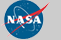Curator: David M. Giles NASA Official: Brent N. Holben Generated: 13/12/2019